# ML Aggarwal Solutions for Class 8 Maths Chapter 10 Algebraic Expressions and Identities

ML Aggarwal Solutions for Class 8 Maths Chapter 10 Algebraic Expressions and Identities are given here to make the exam preparations of students easier. This chapter mainly deals with problems based on identifying and performing operations on algebraic expressions and identities. Students are provided with simple step-by-step solutions in order to make them comprehend concepts clearly. Our experts in the subject have created these solutions which include tips and tricks for better understanding. It’s highly recommended for students aspiring to secure high marks in their examination to practice these solutions on a regular basis. ML Aggarwal Solutions PDF of this chapter can be downloaded from the link provided below and can be used for any future references.

Chapter 10 Algebraic Expressions and Identities contains five exercises and Check your progress questions. The ML Aggarwal Class 8 Solutions present on this page provides clear solutions, according to the latest ICSE guidelines, so that students can score high marks in the examinations.

## Download the PDF of ML Aggarwal Solutions for Class 8 Maths Chapter 10 Algebraic Expressions and Identities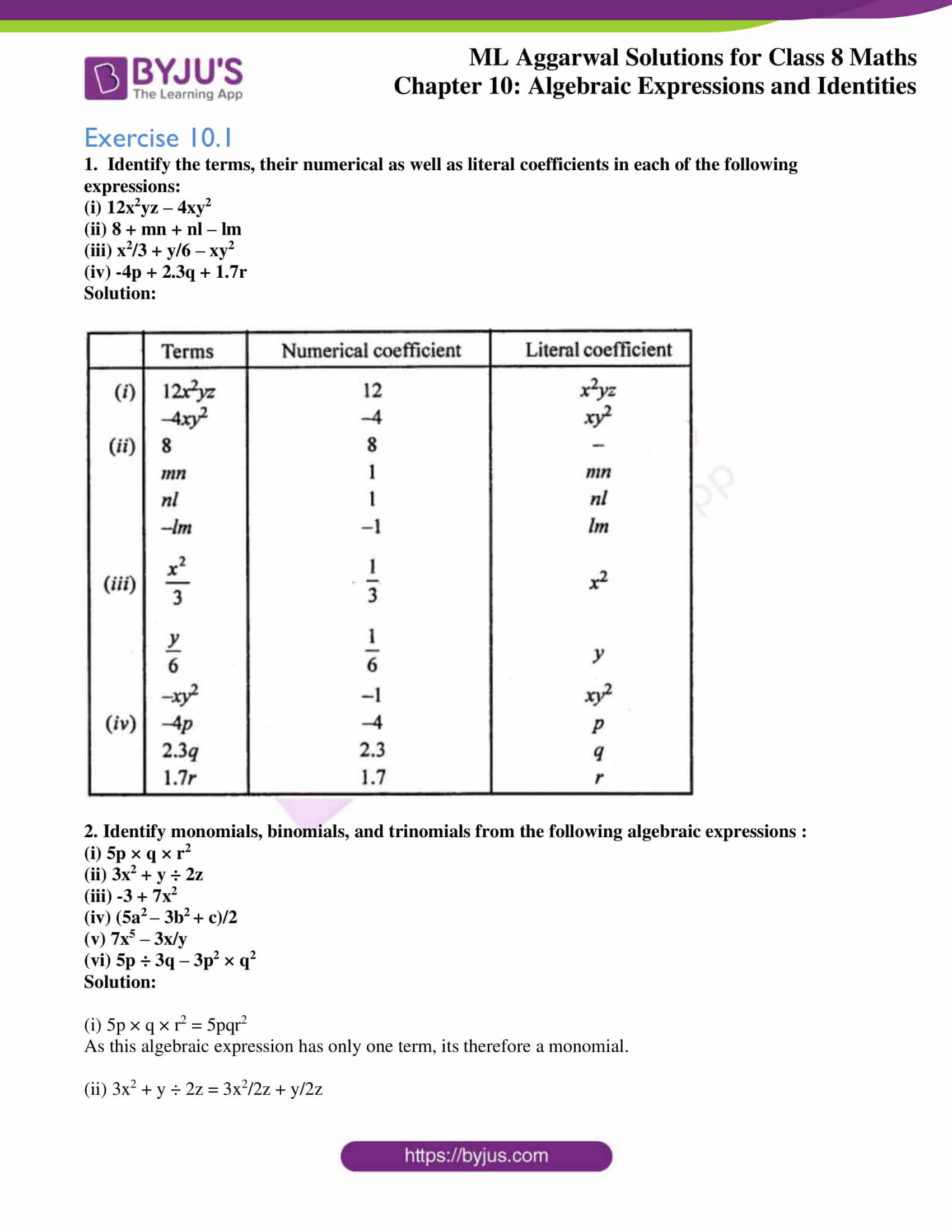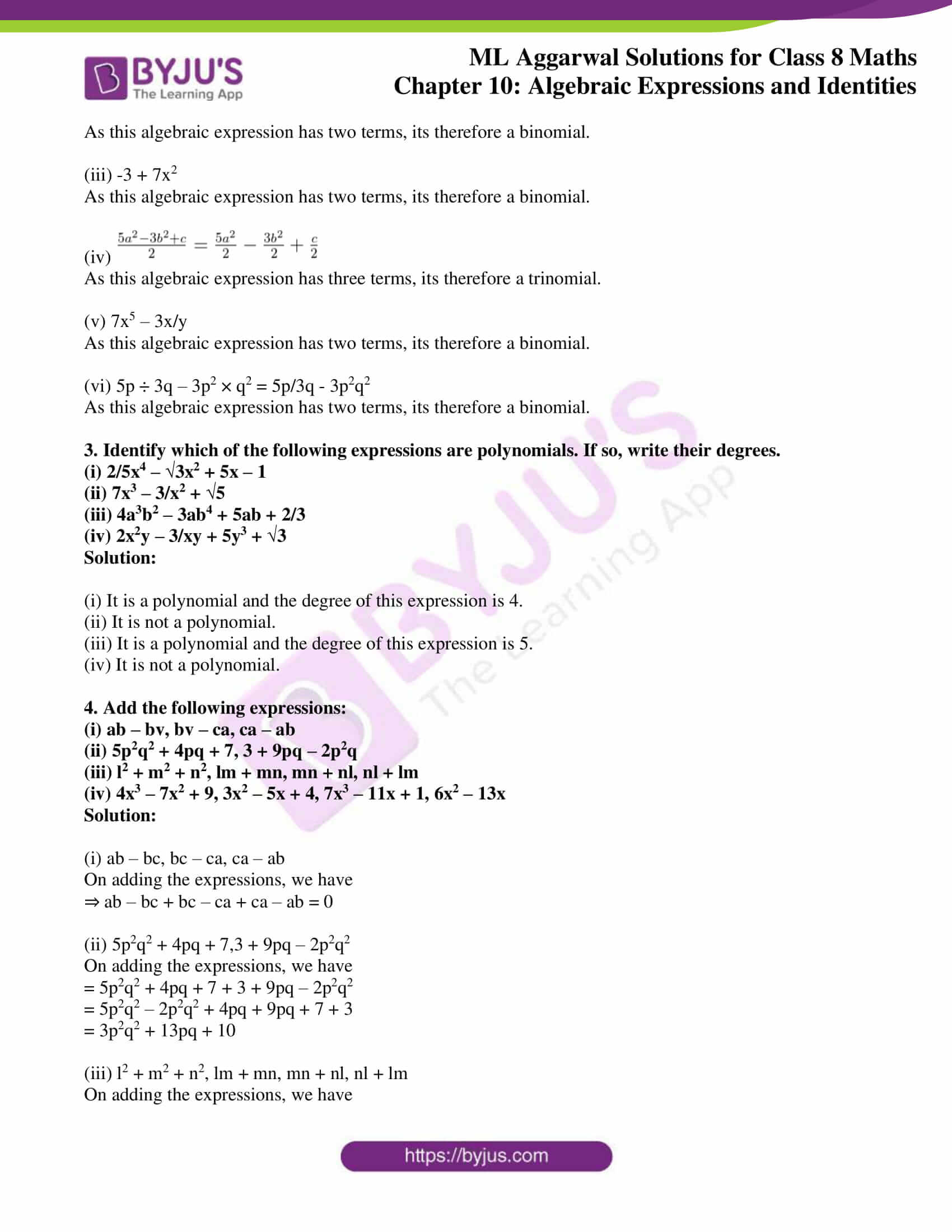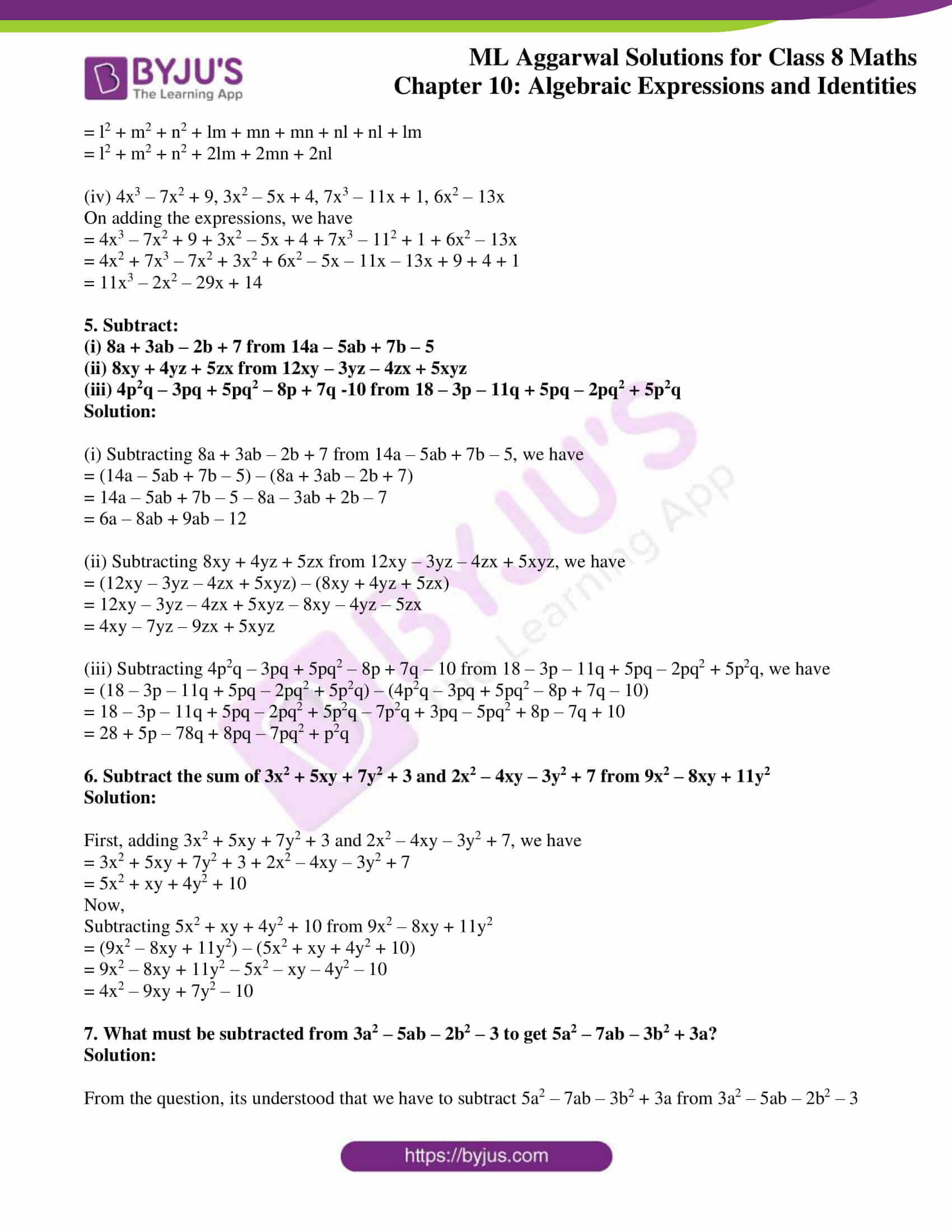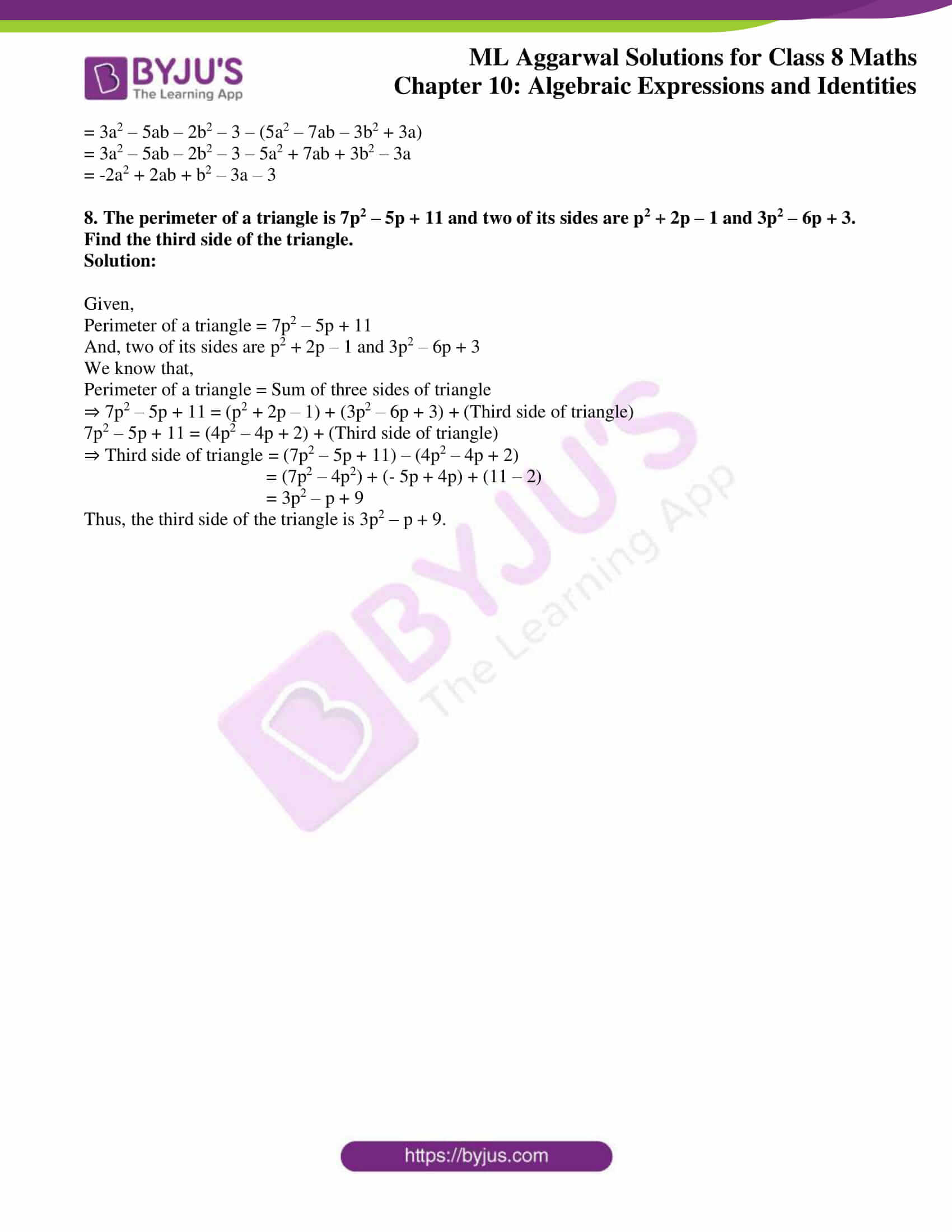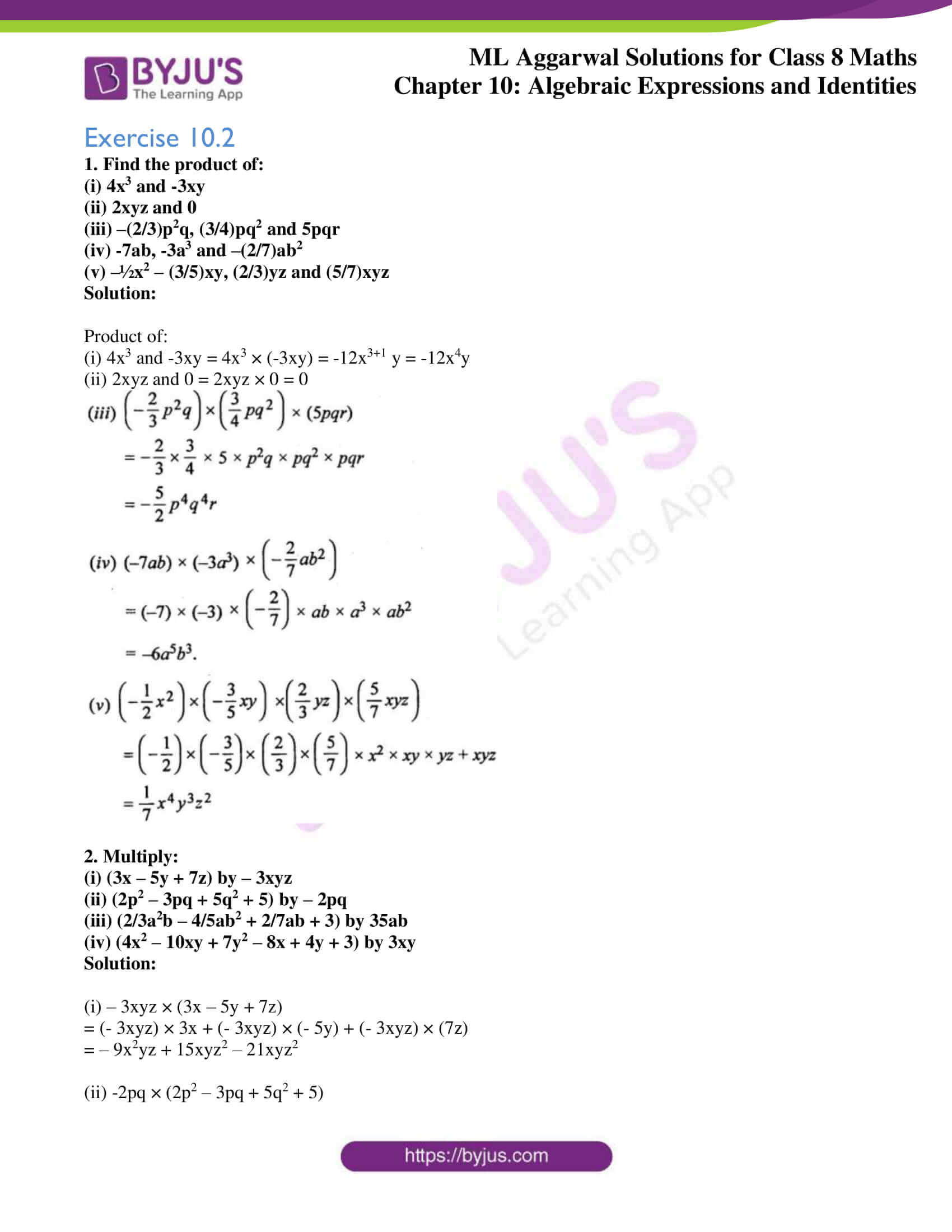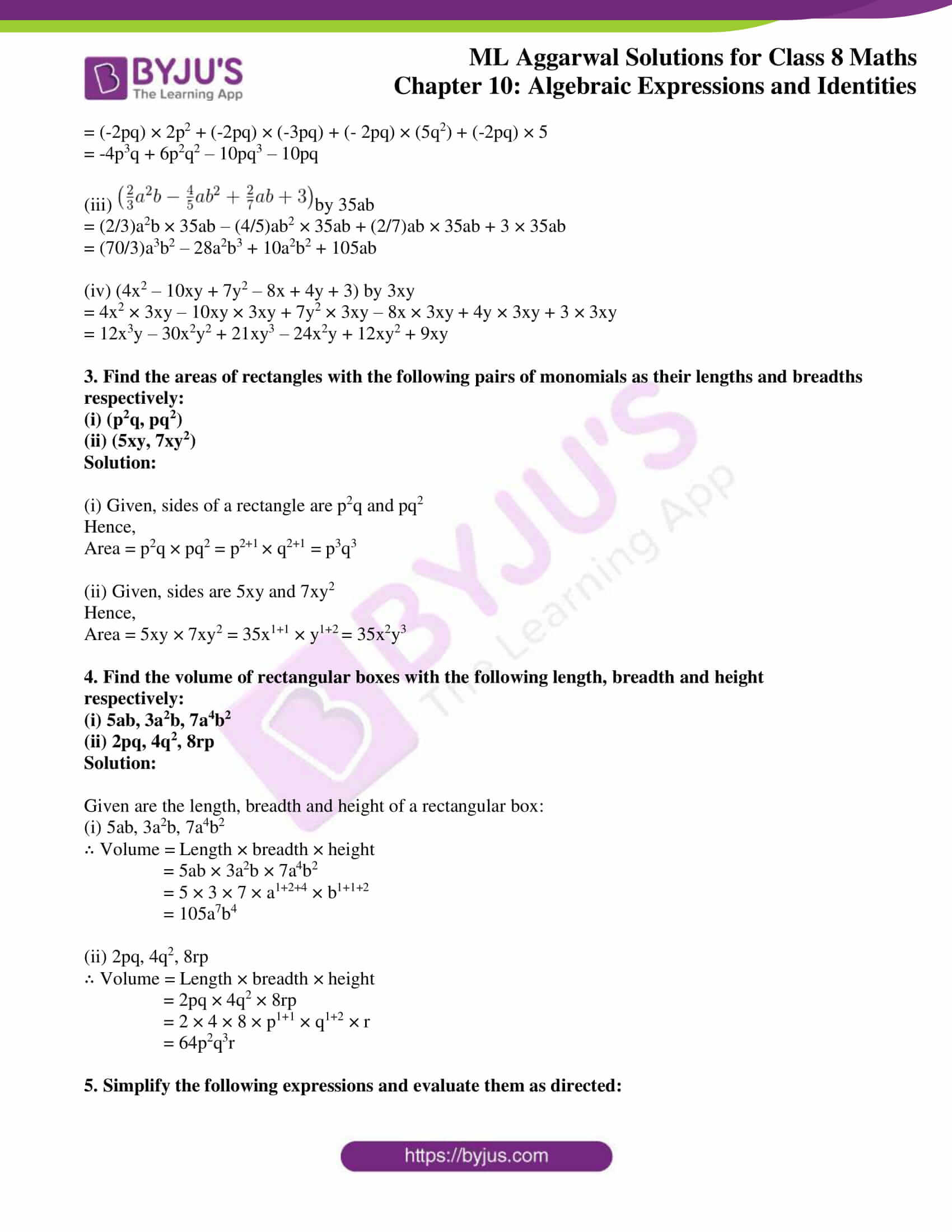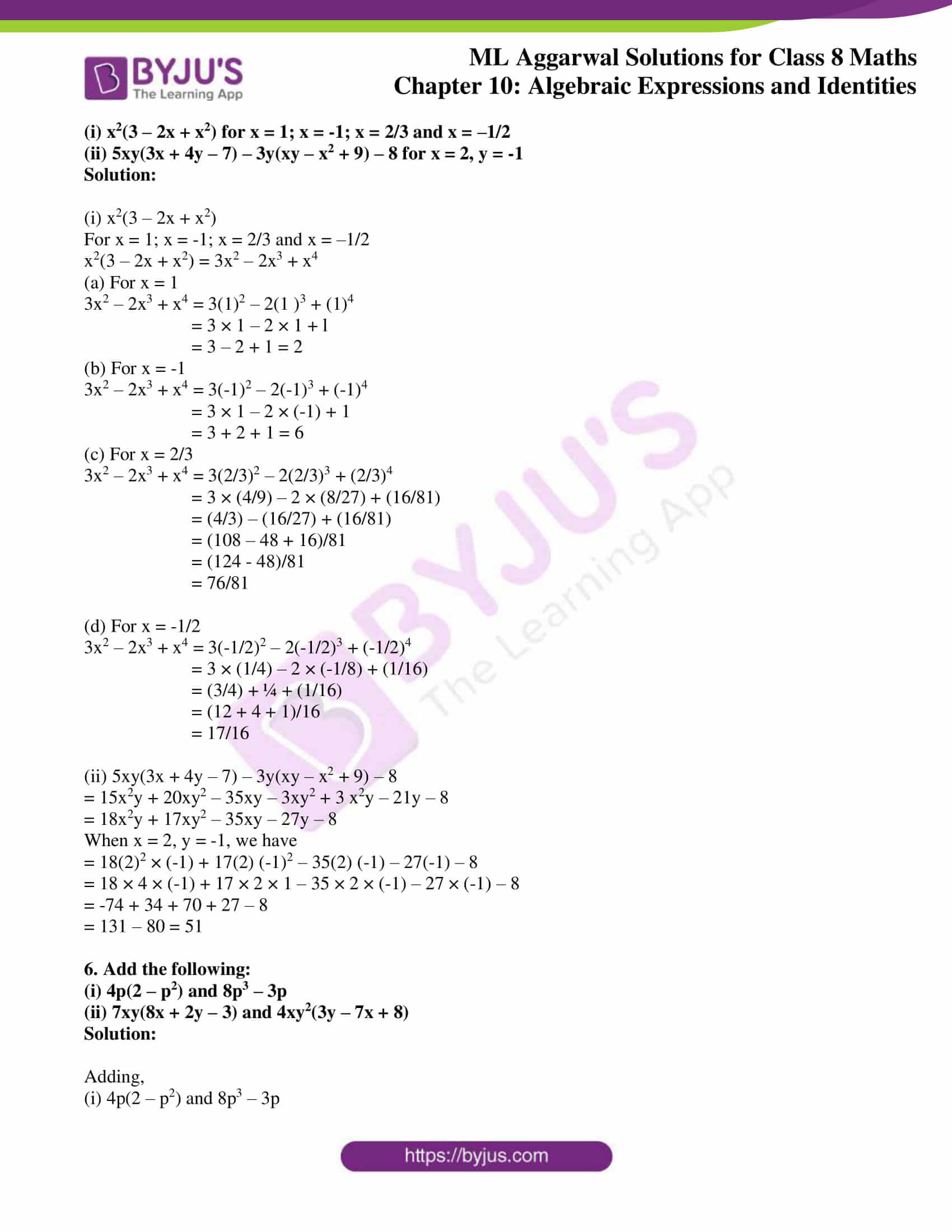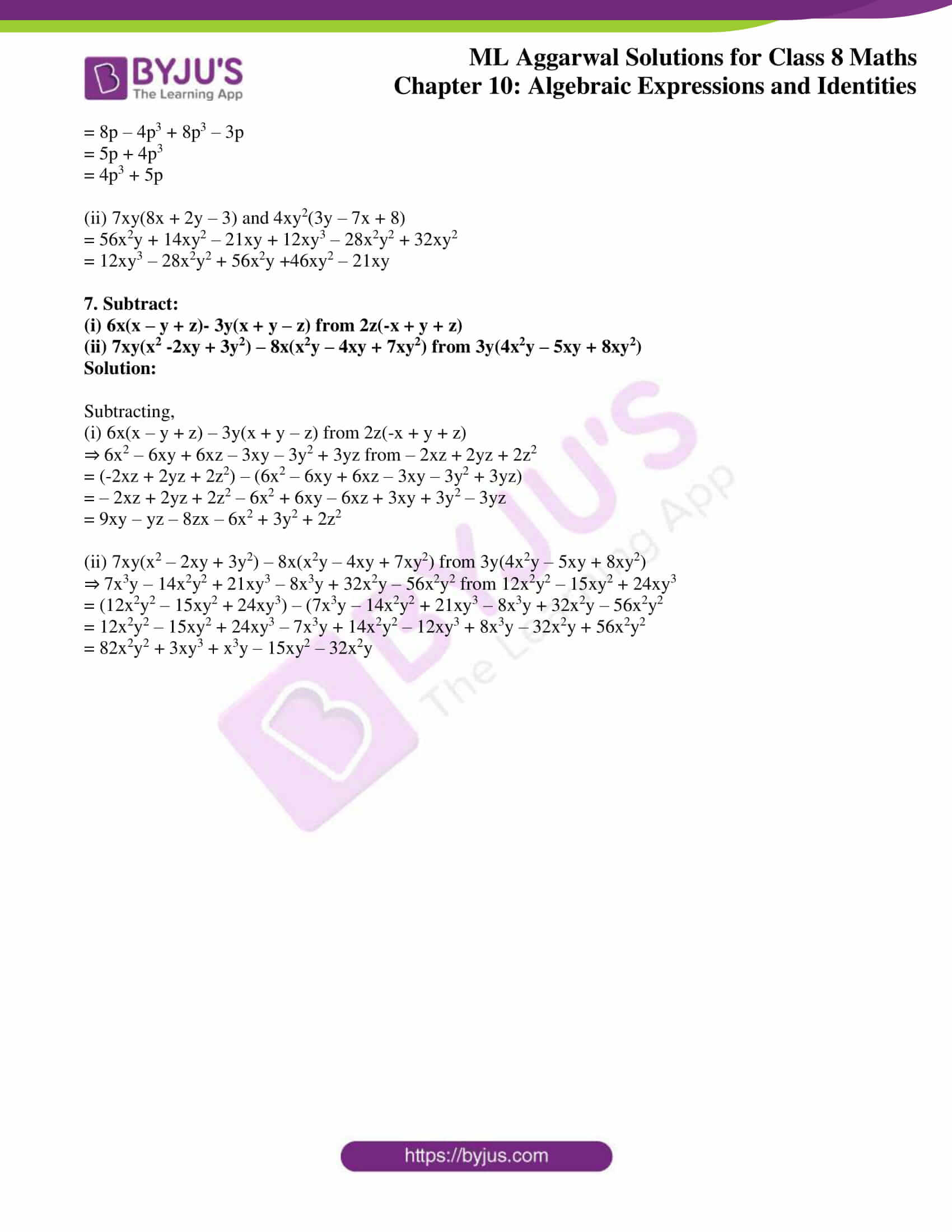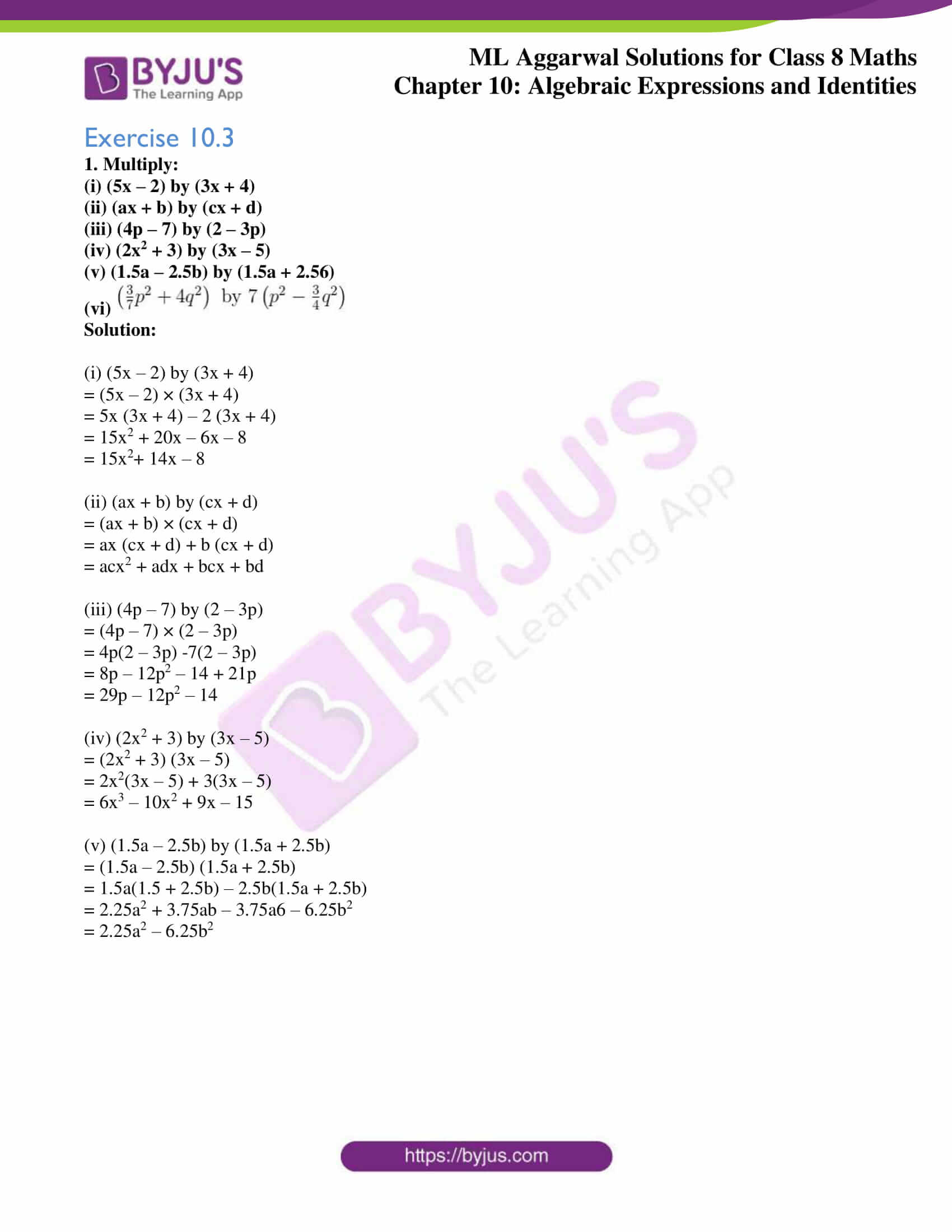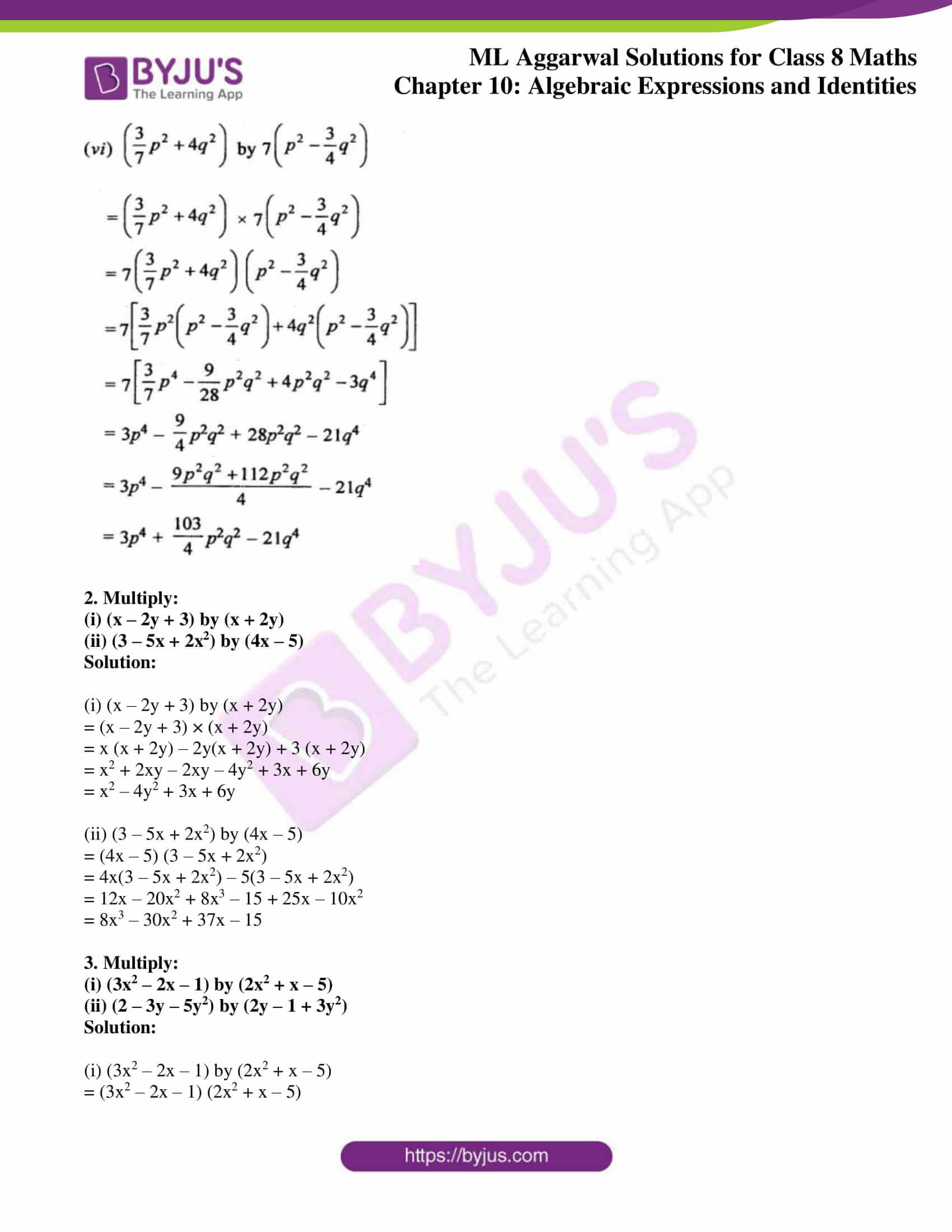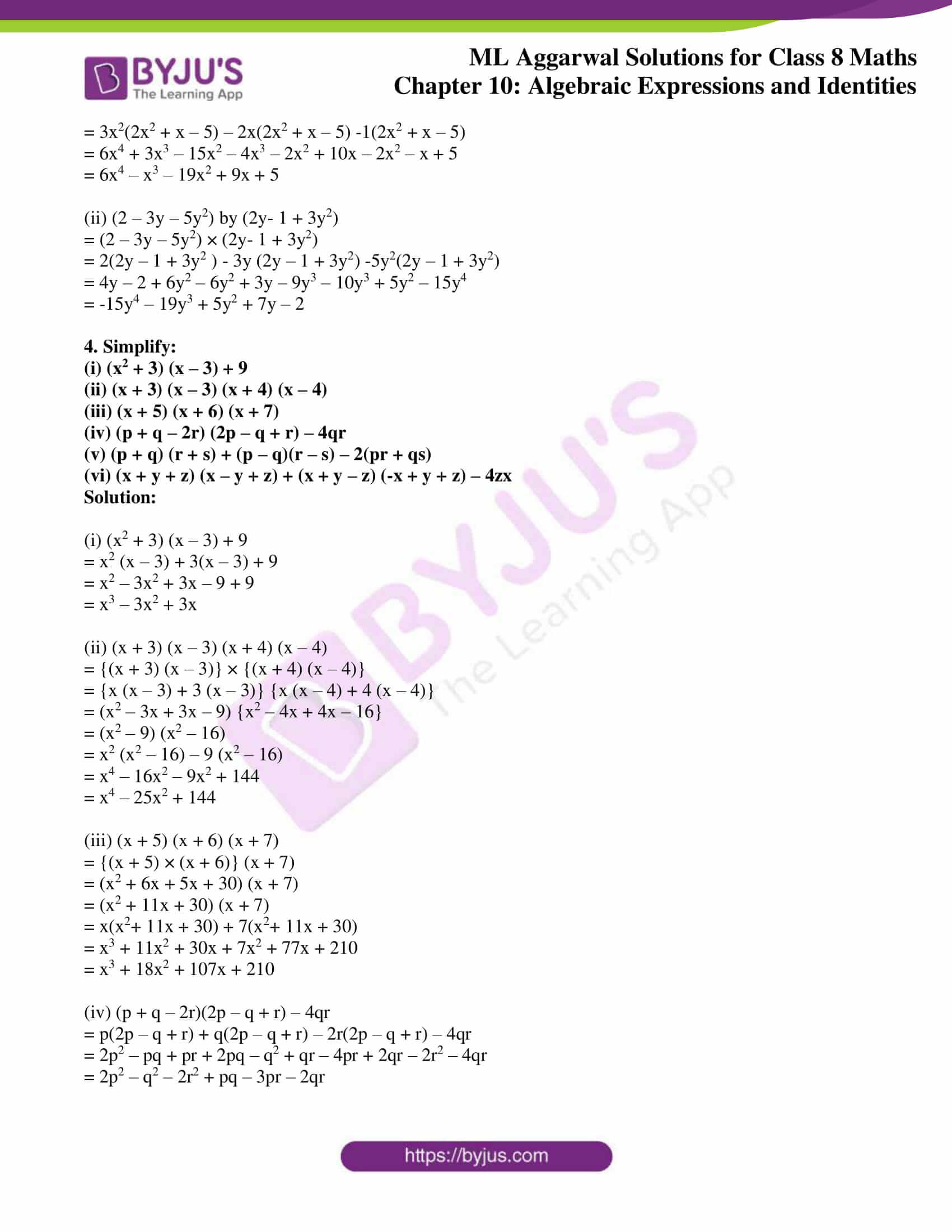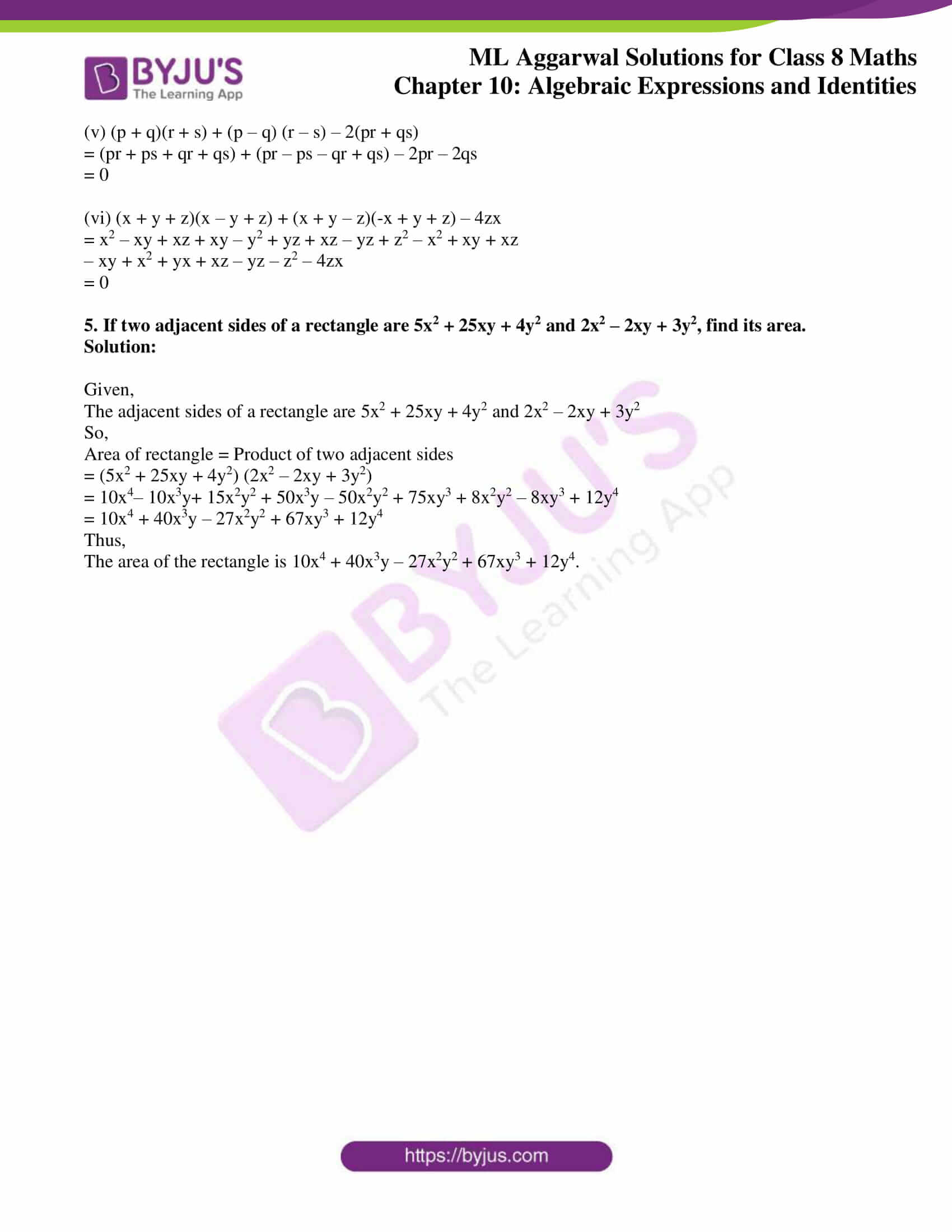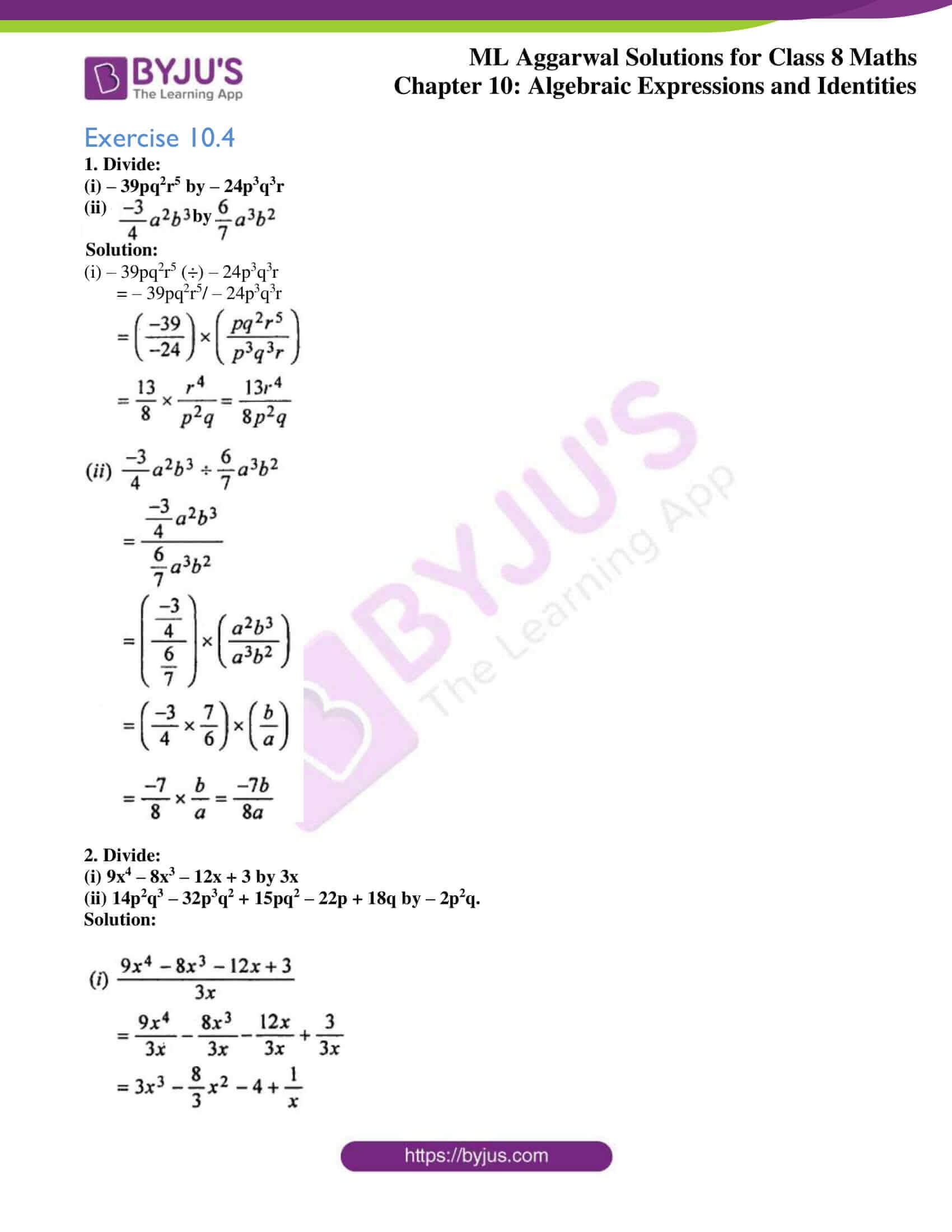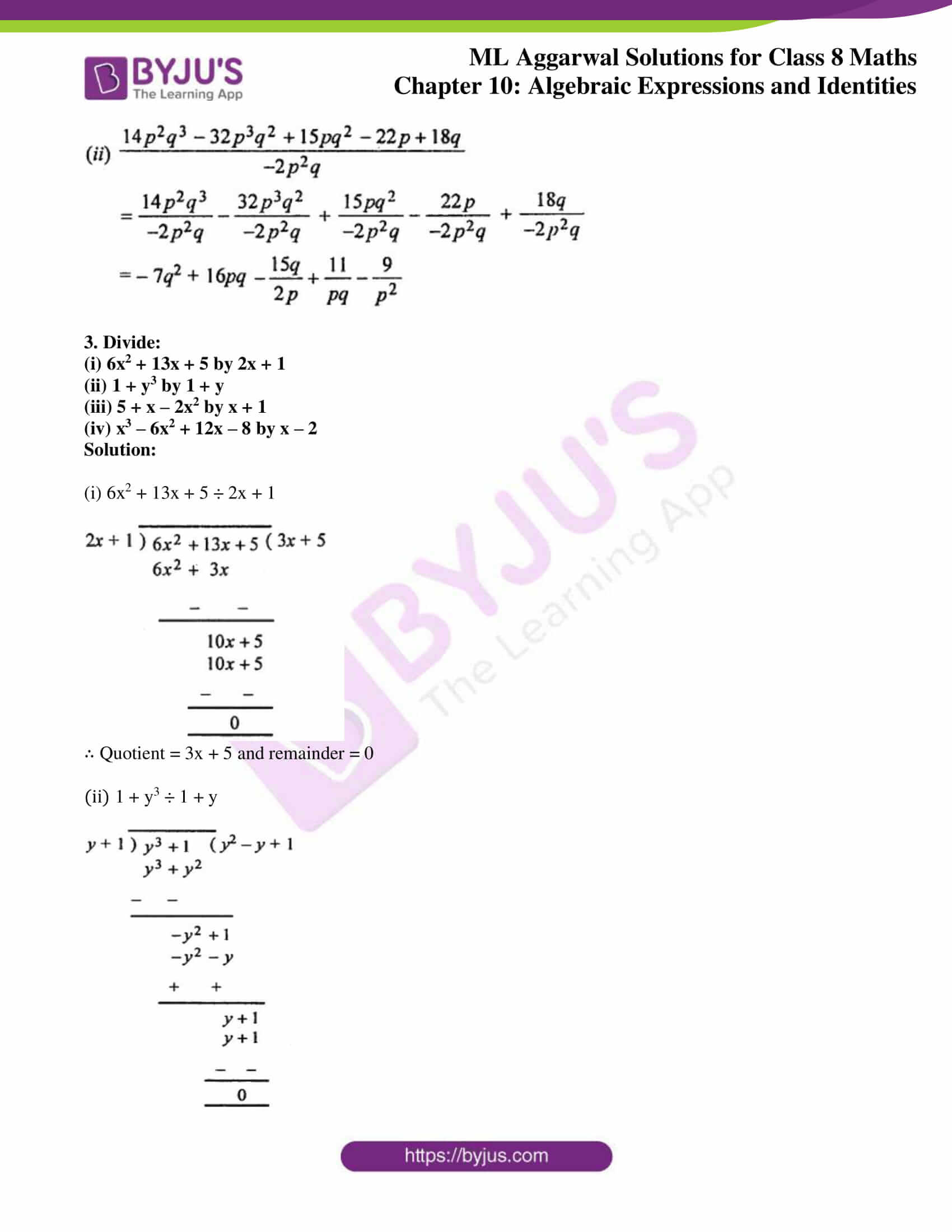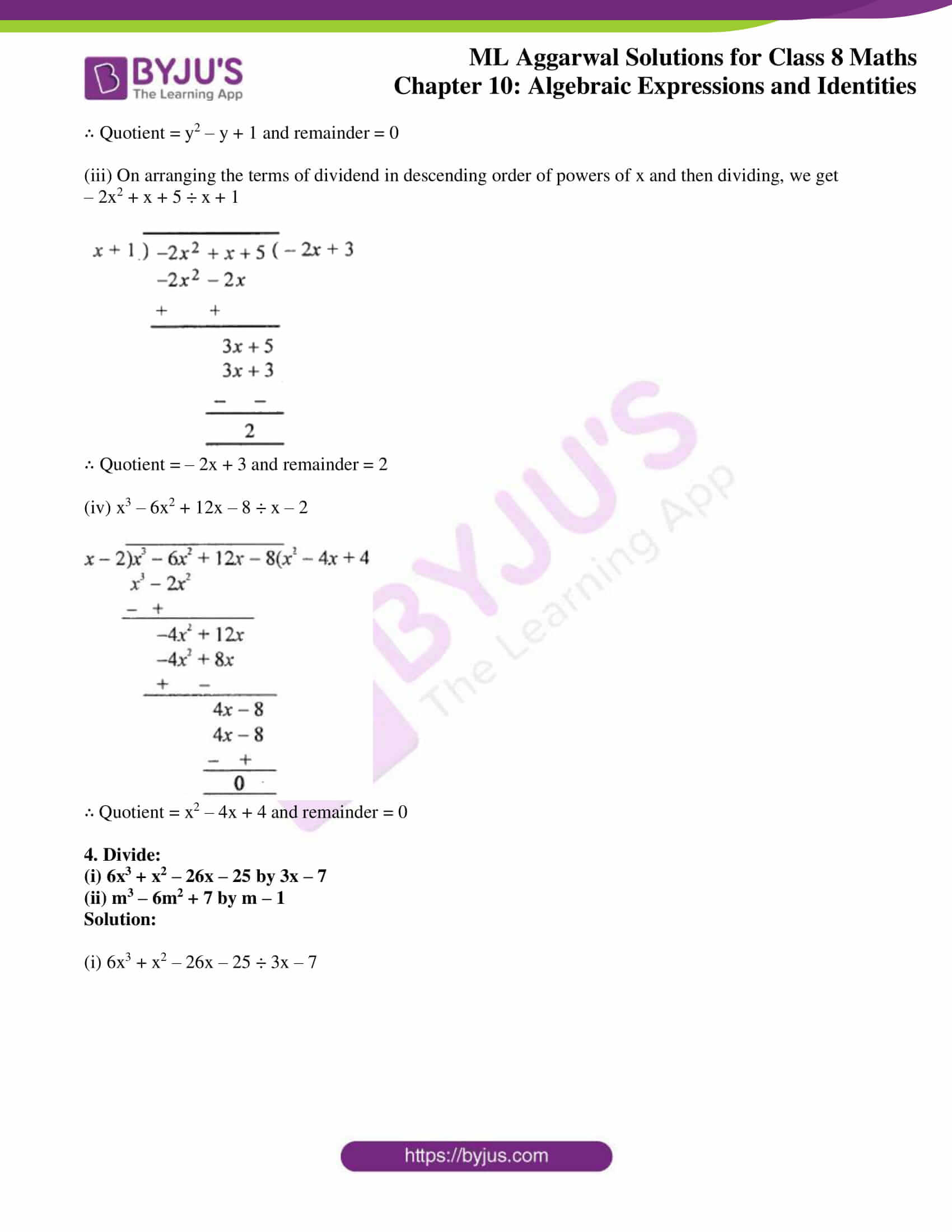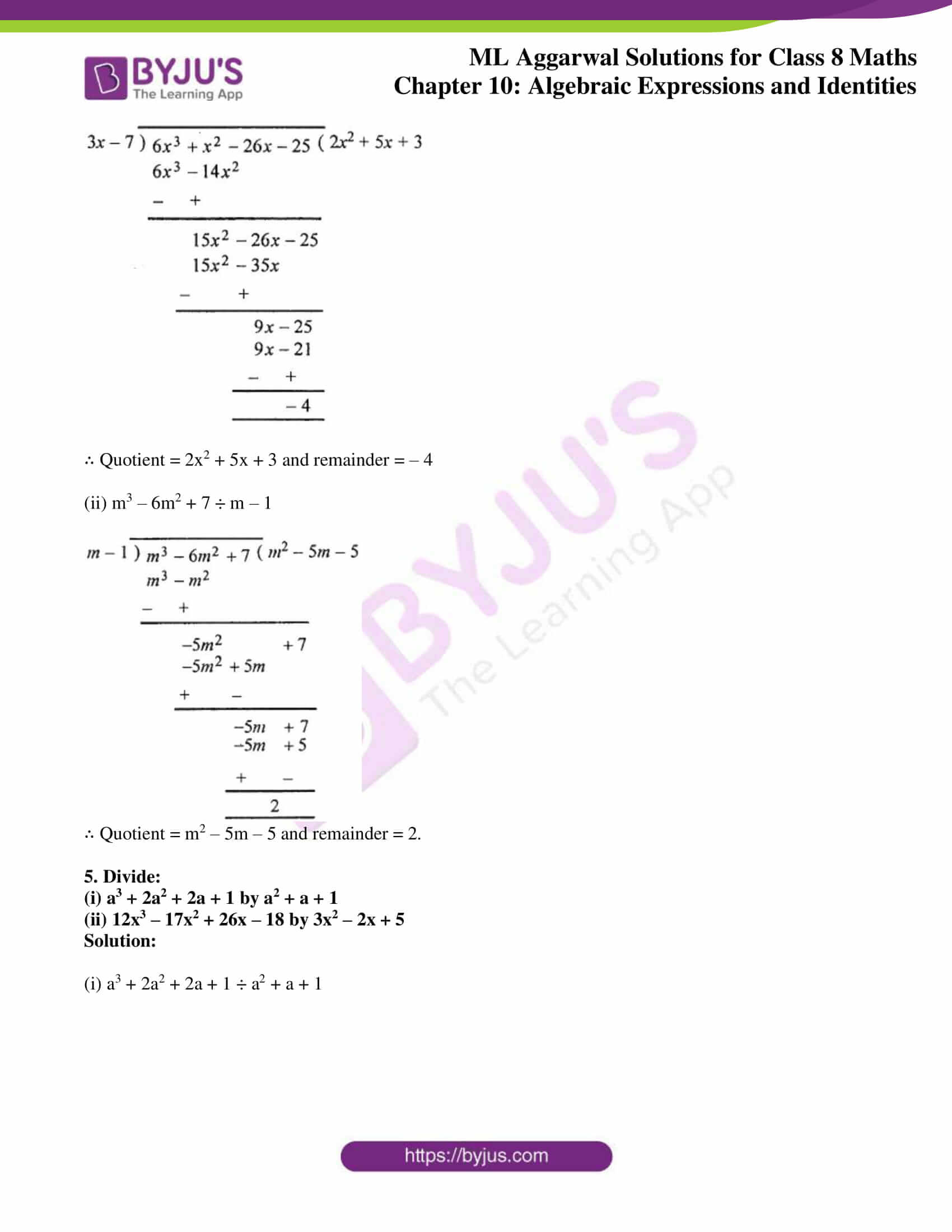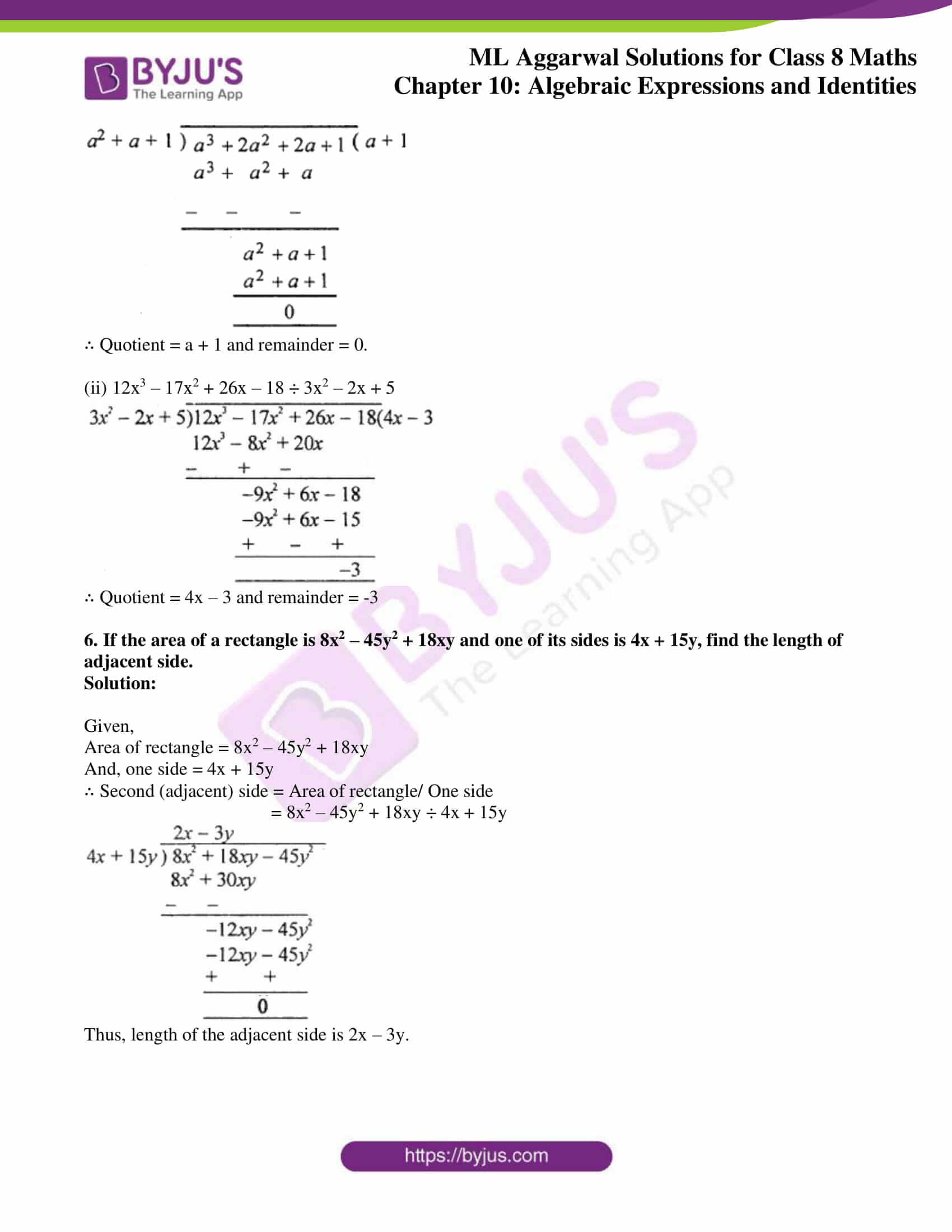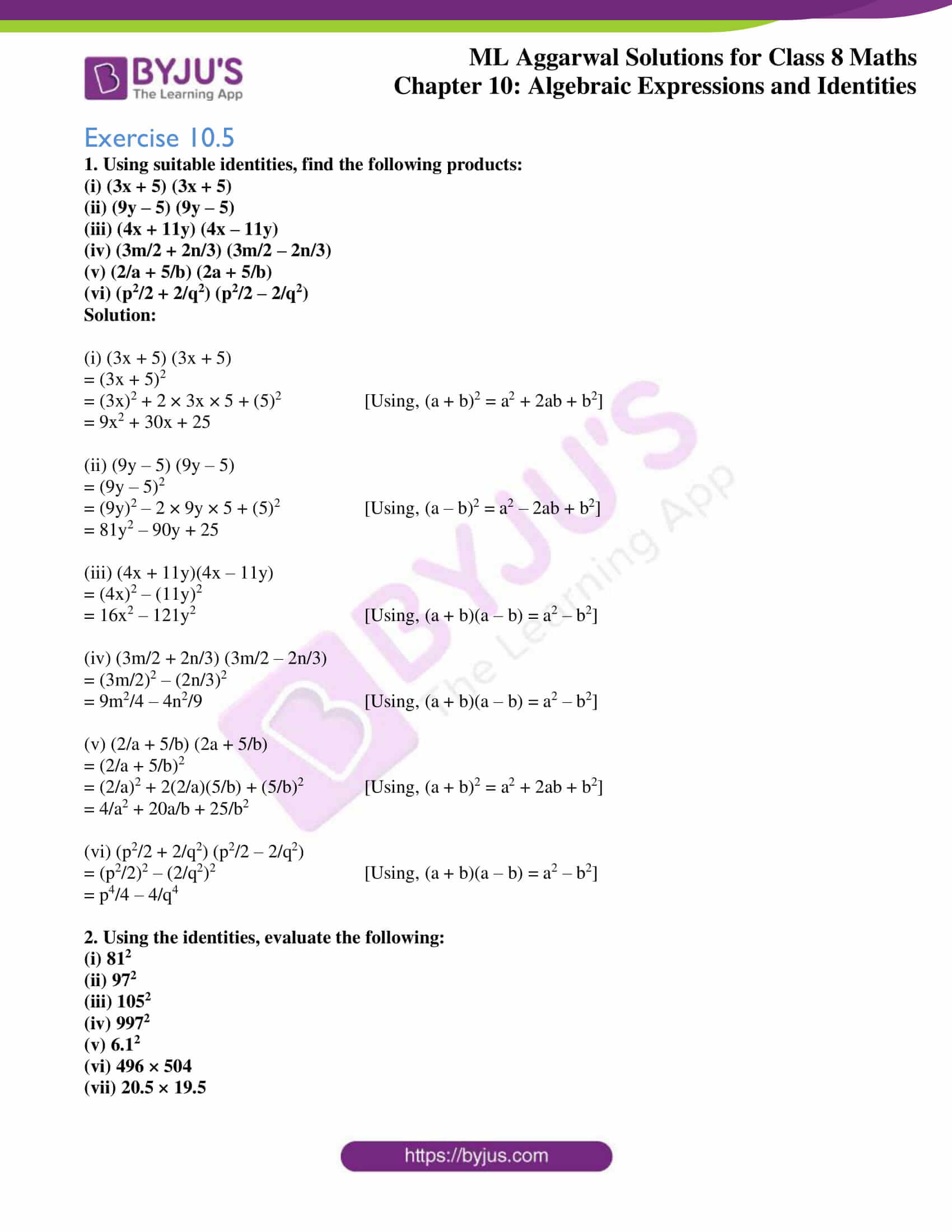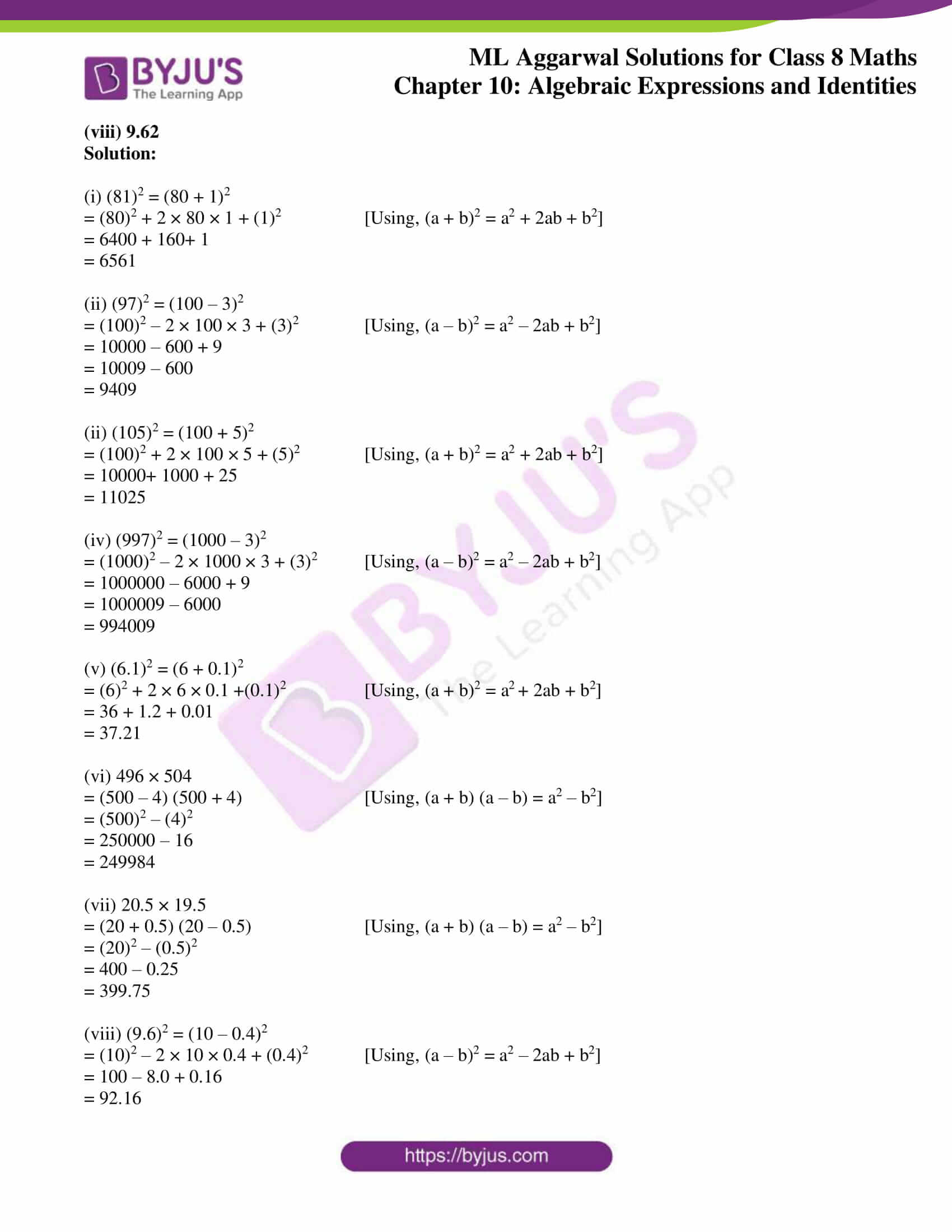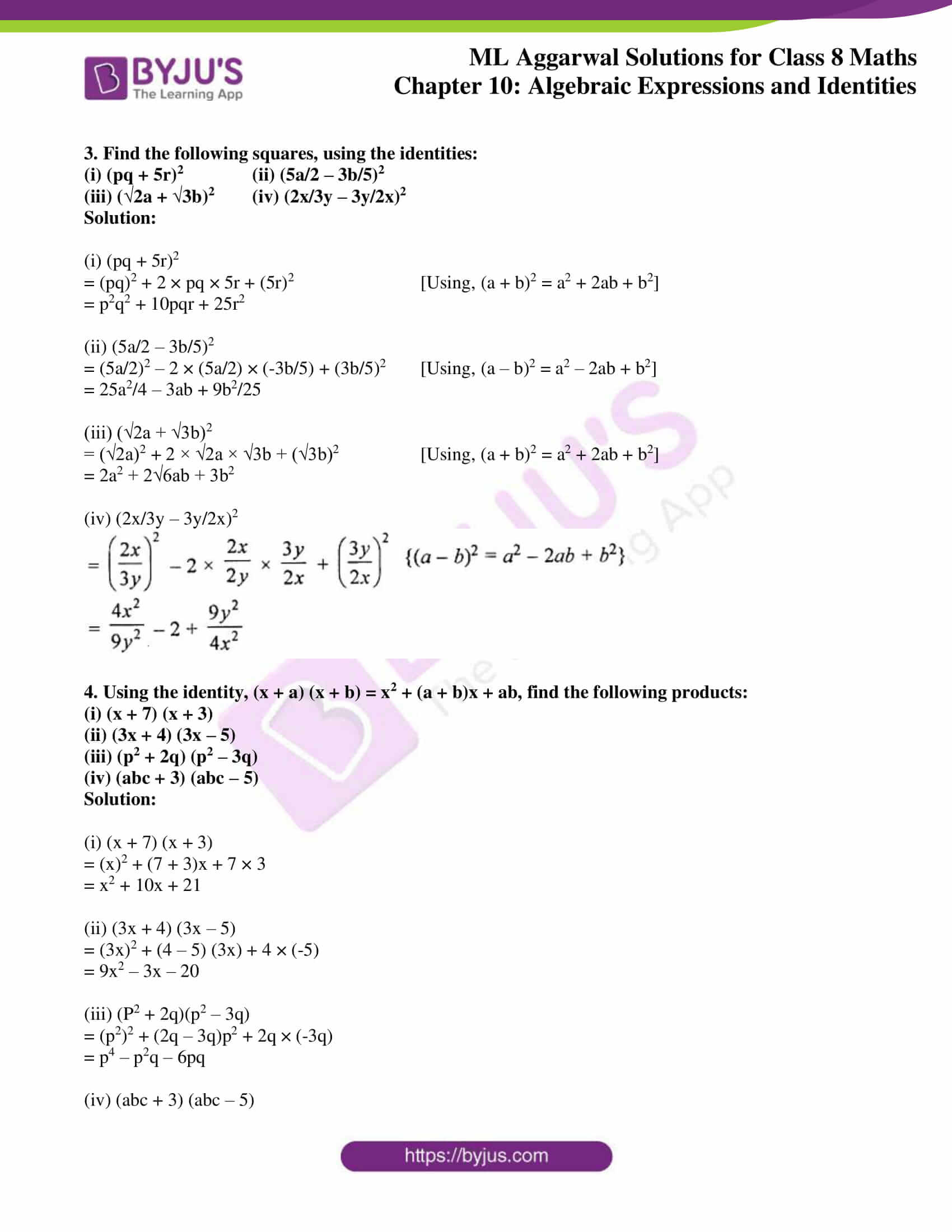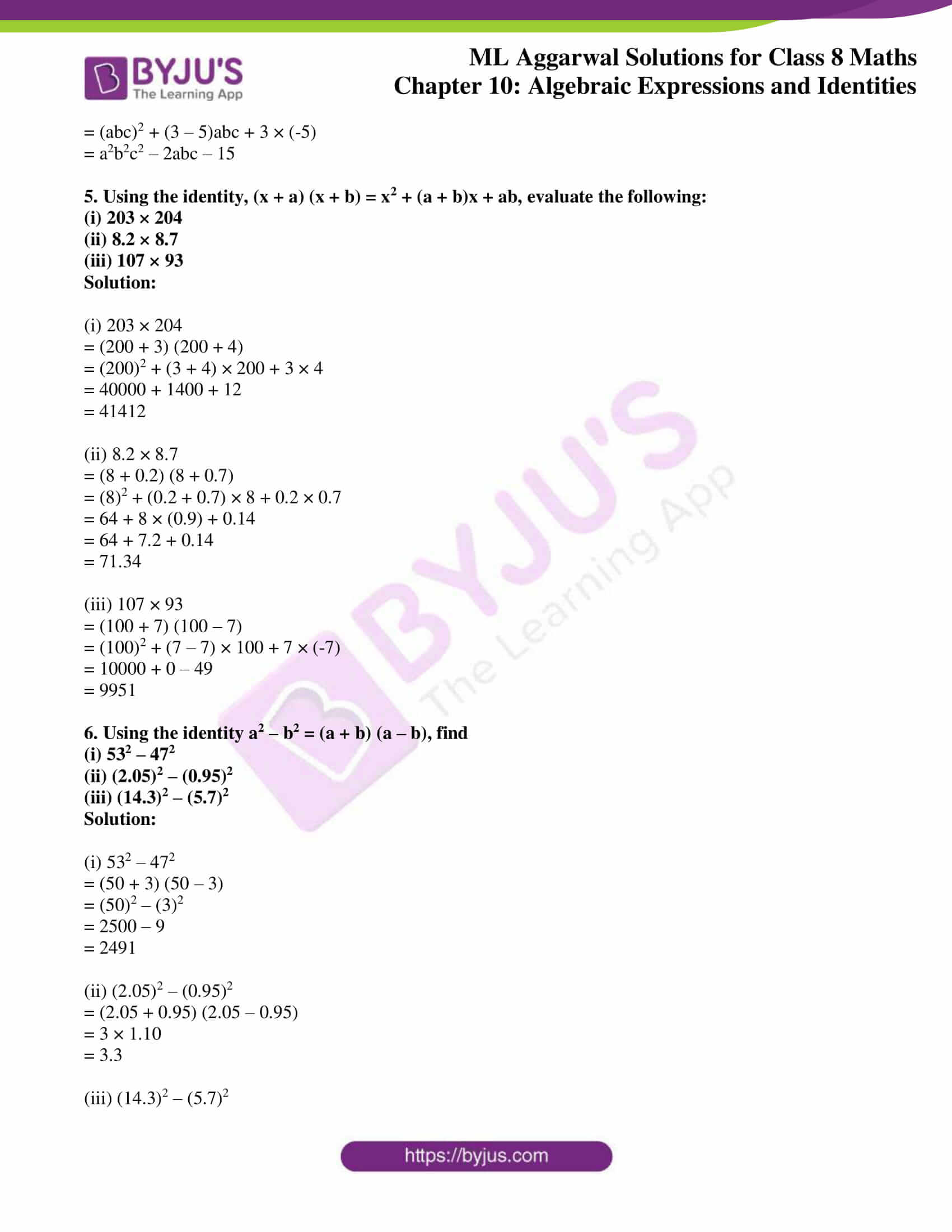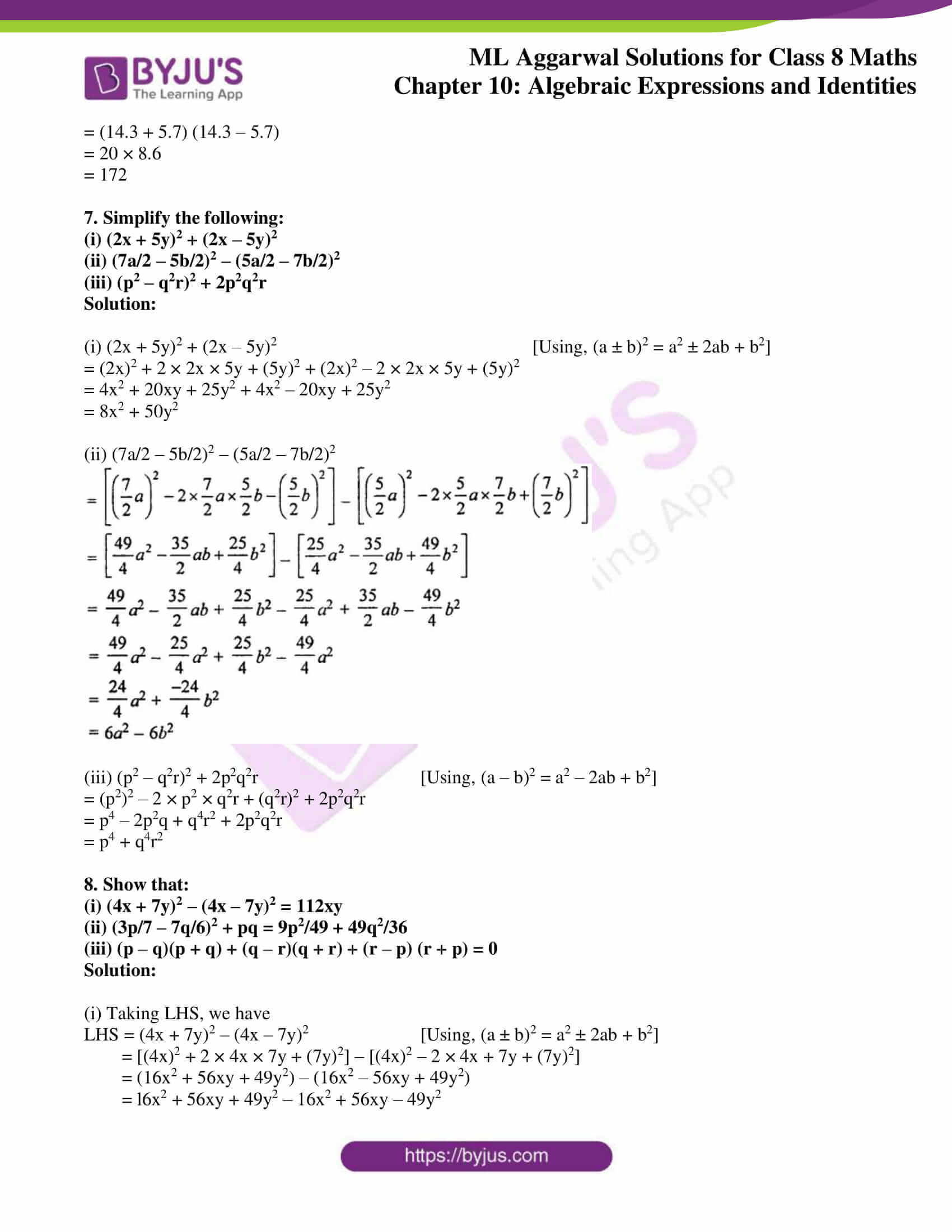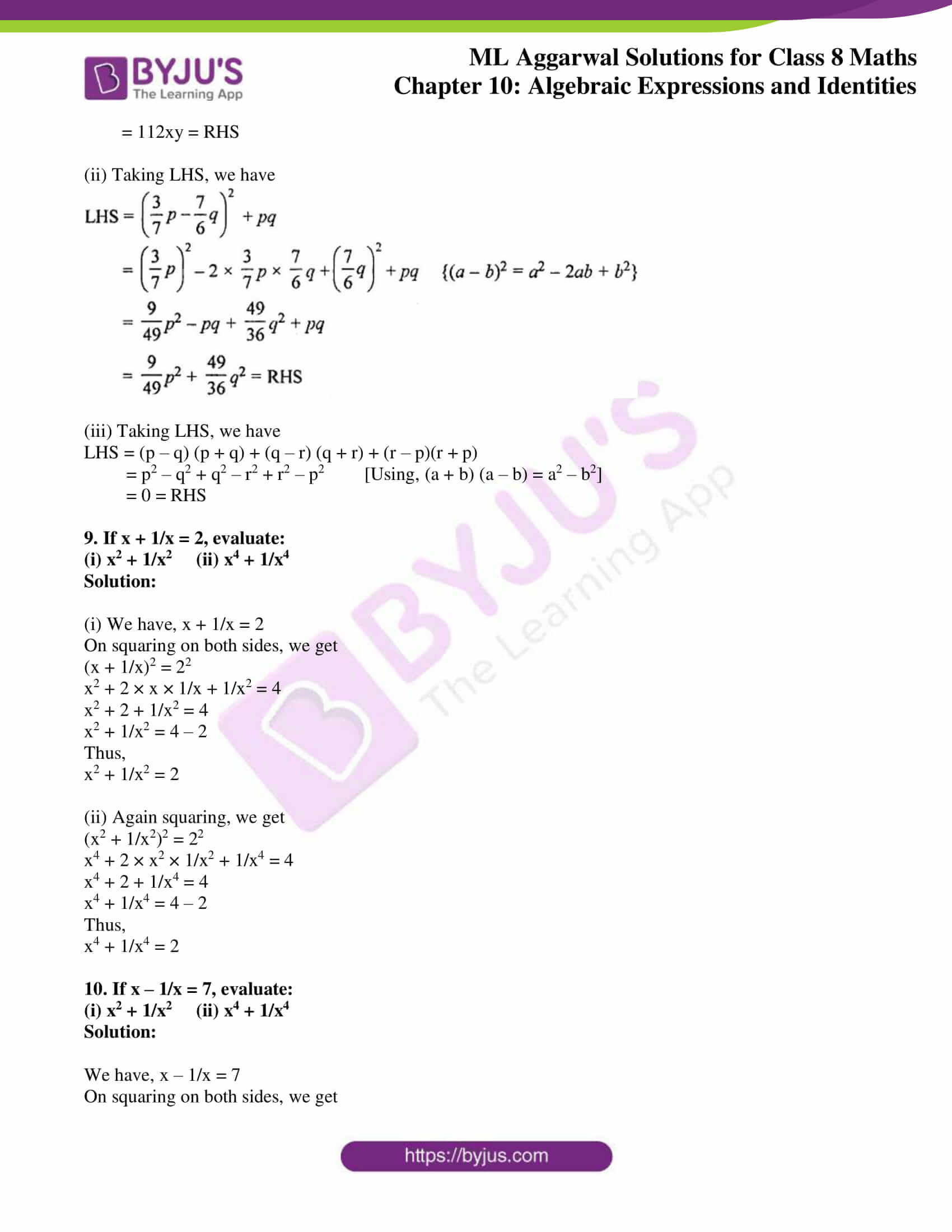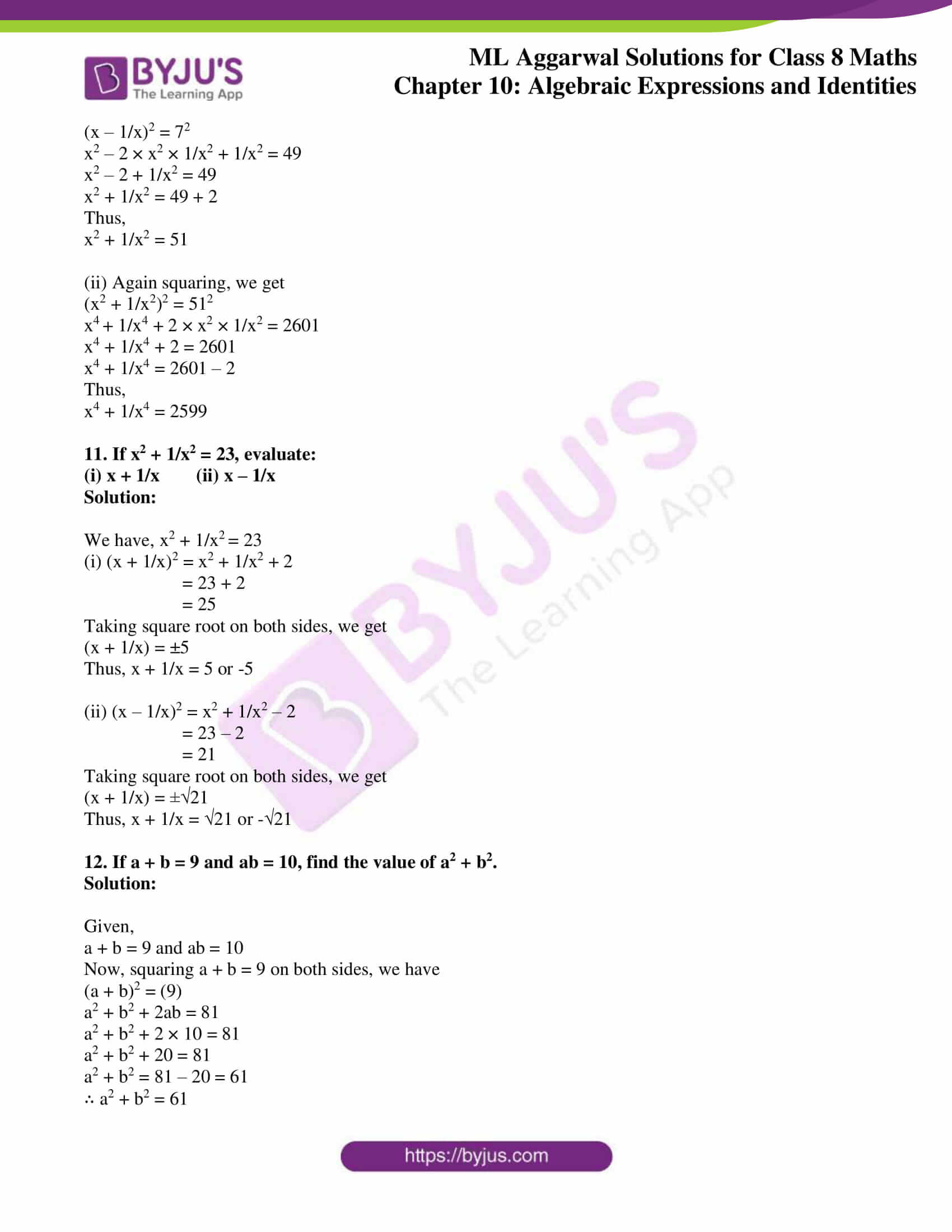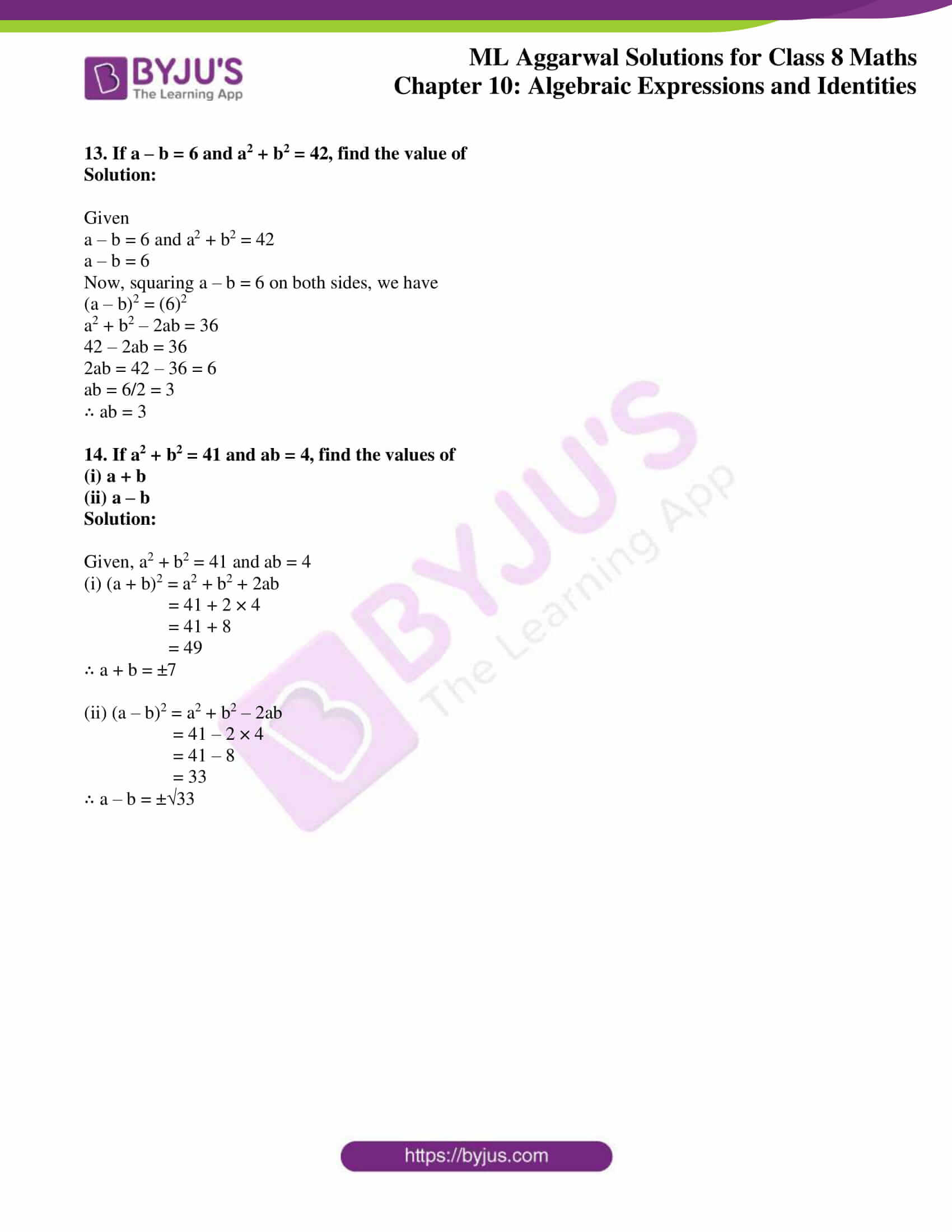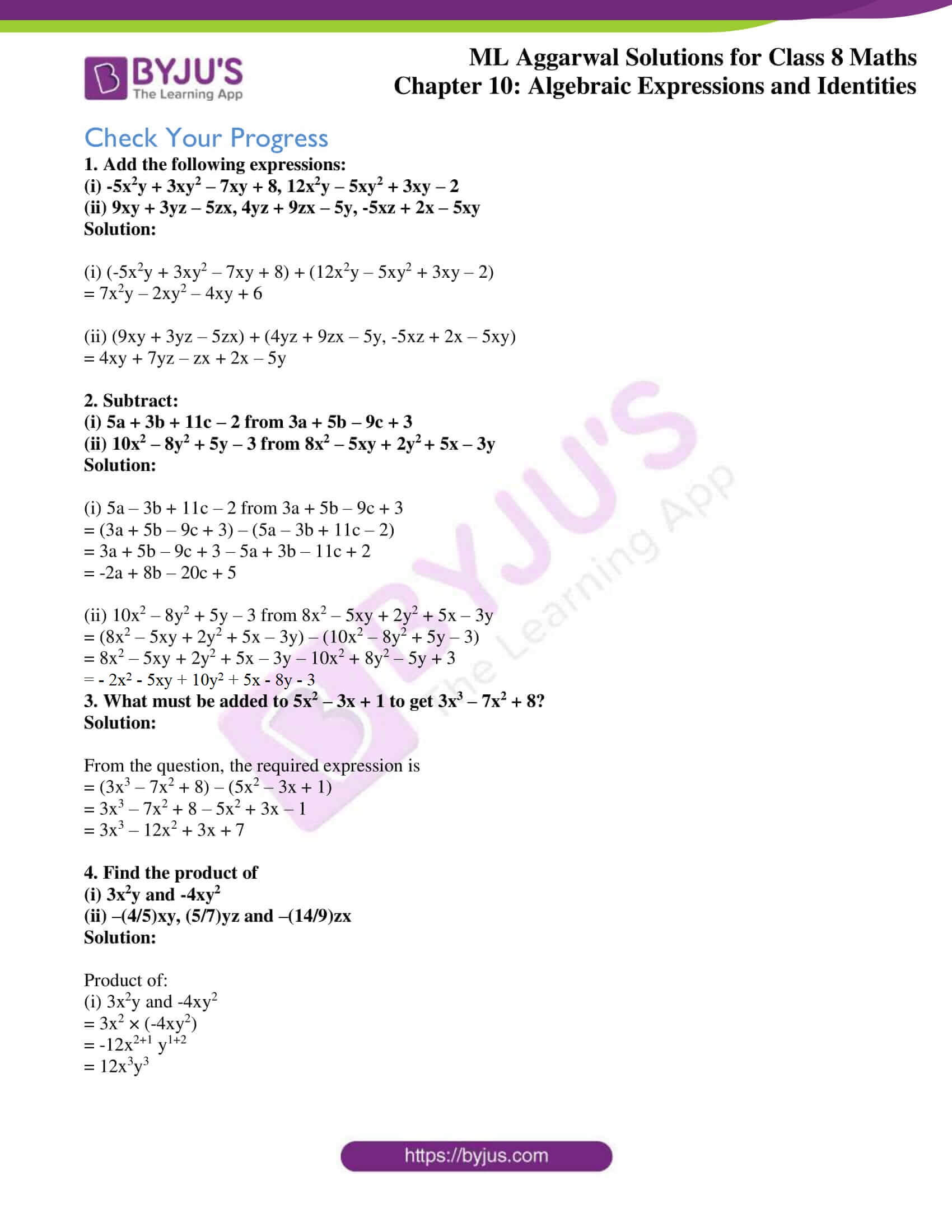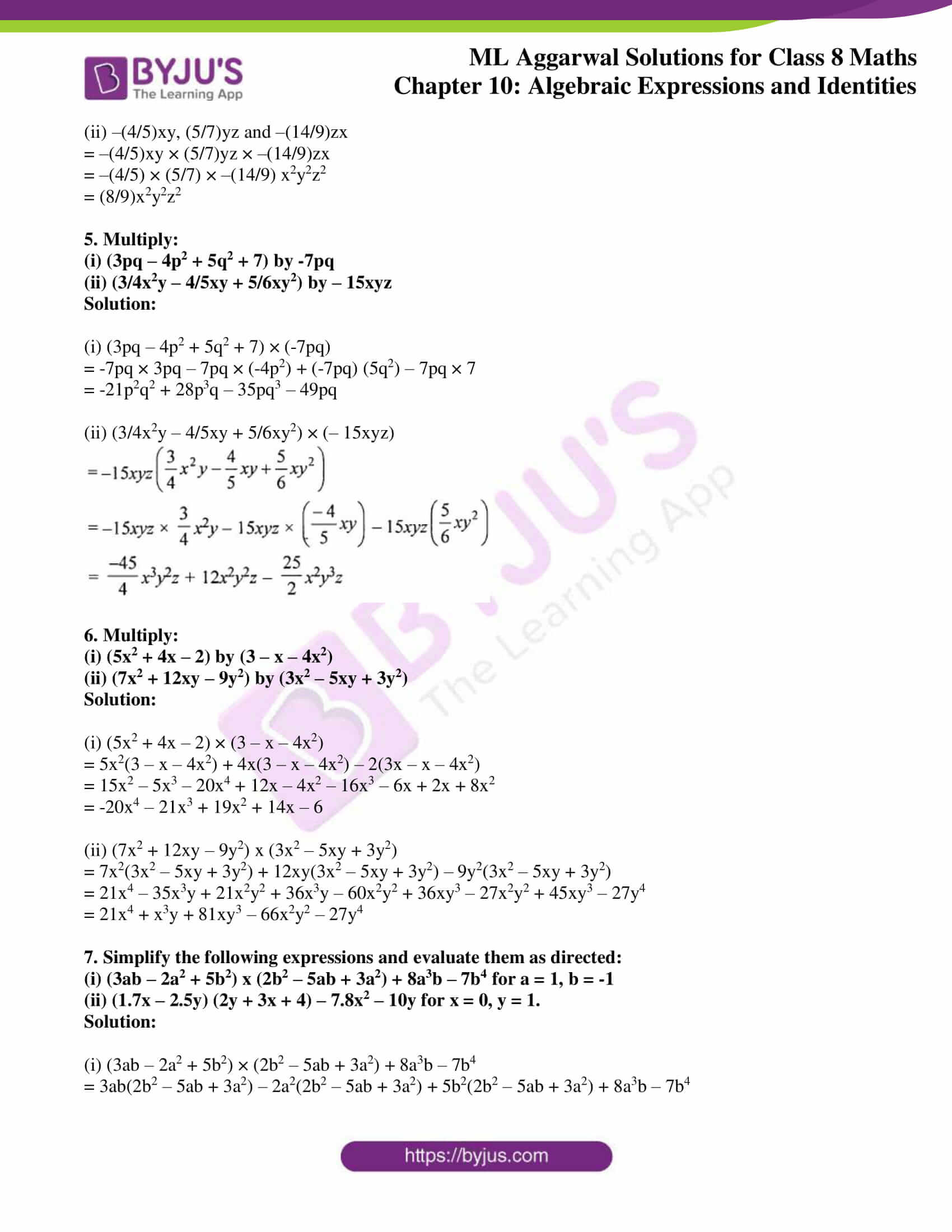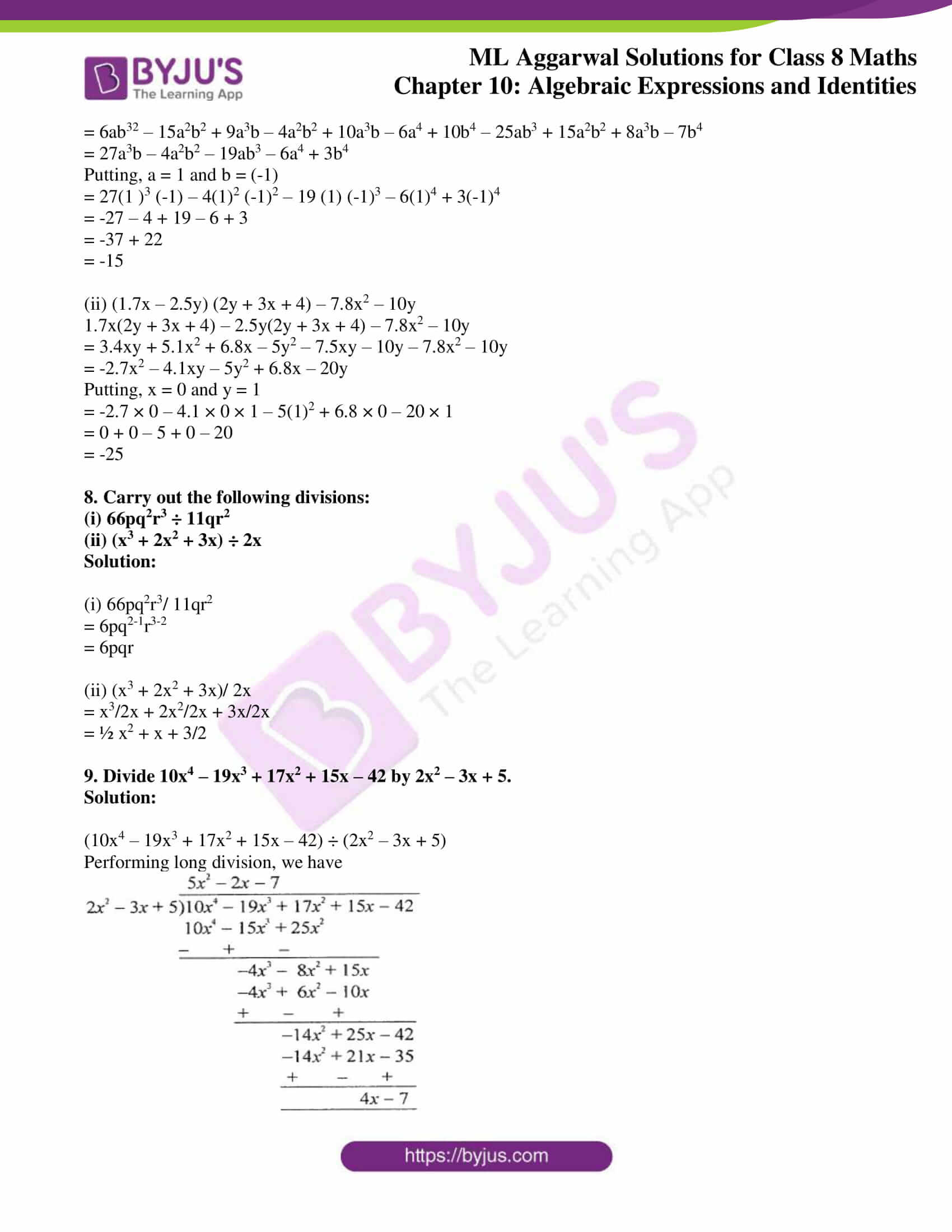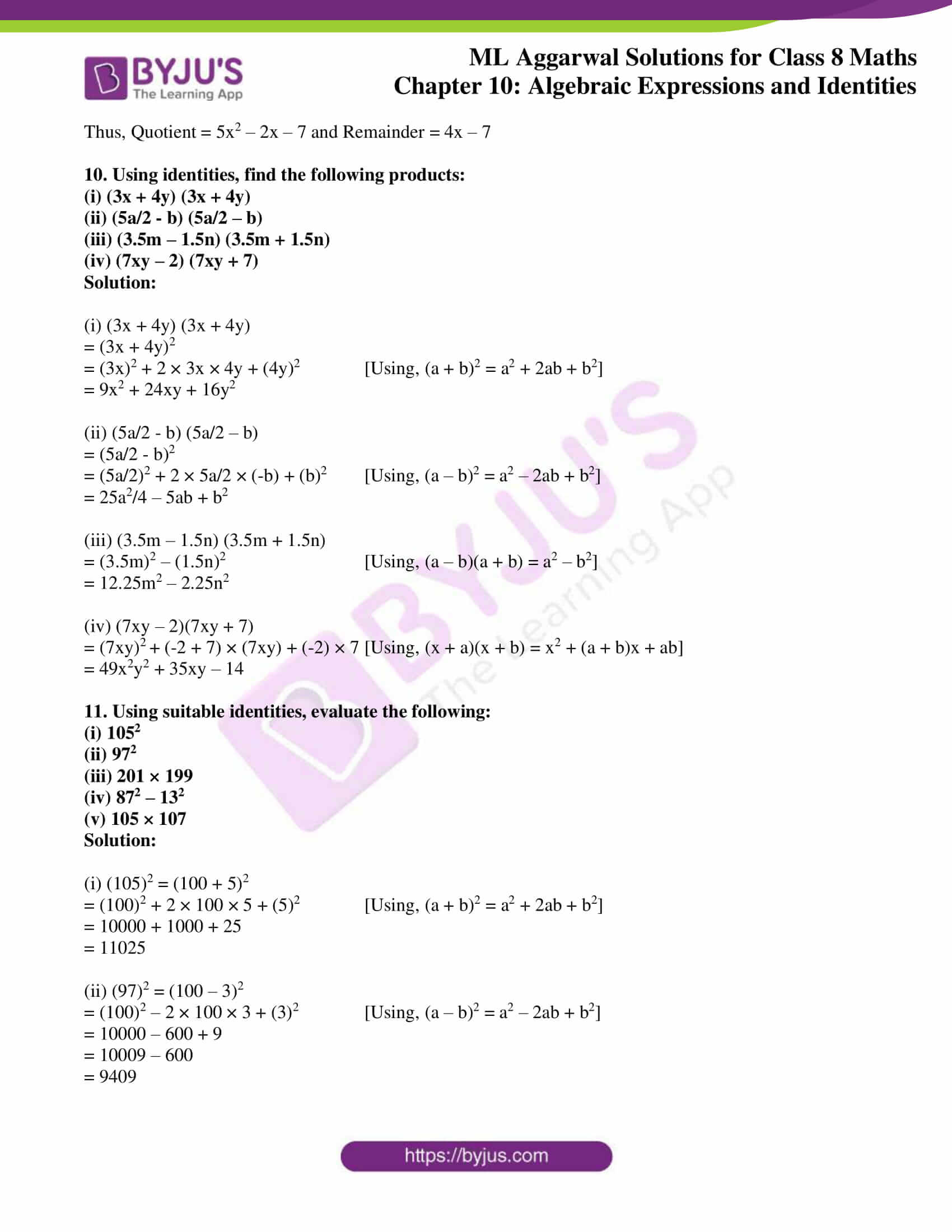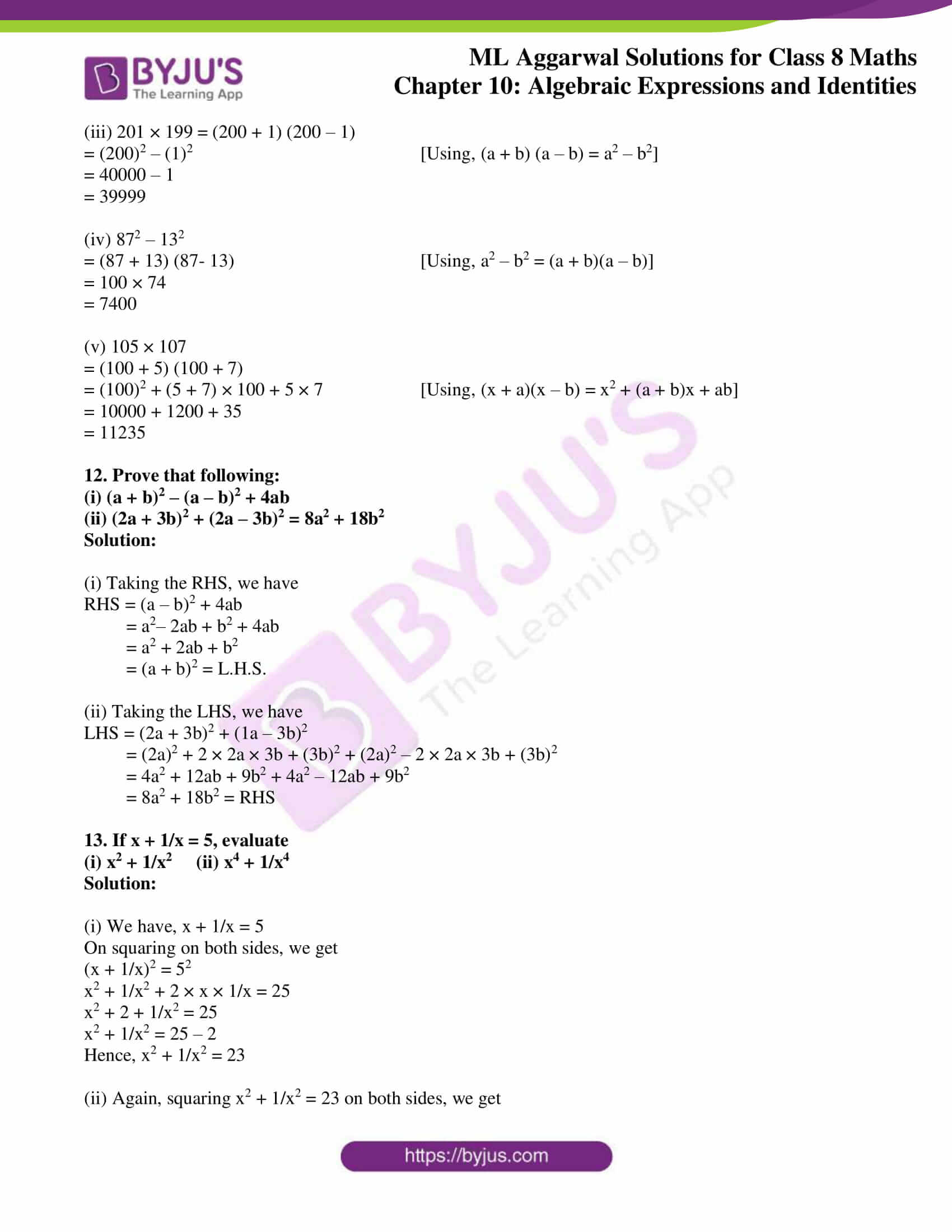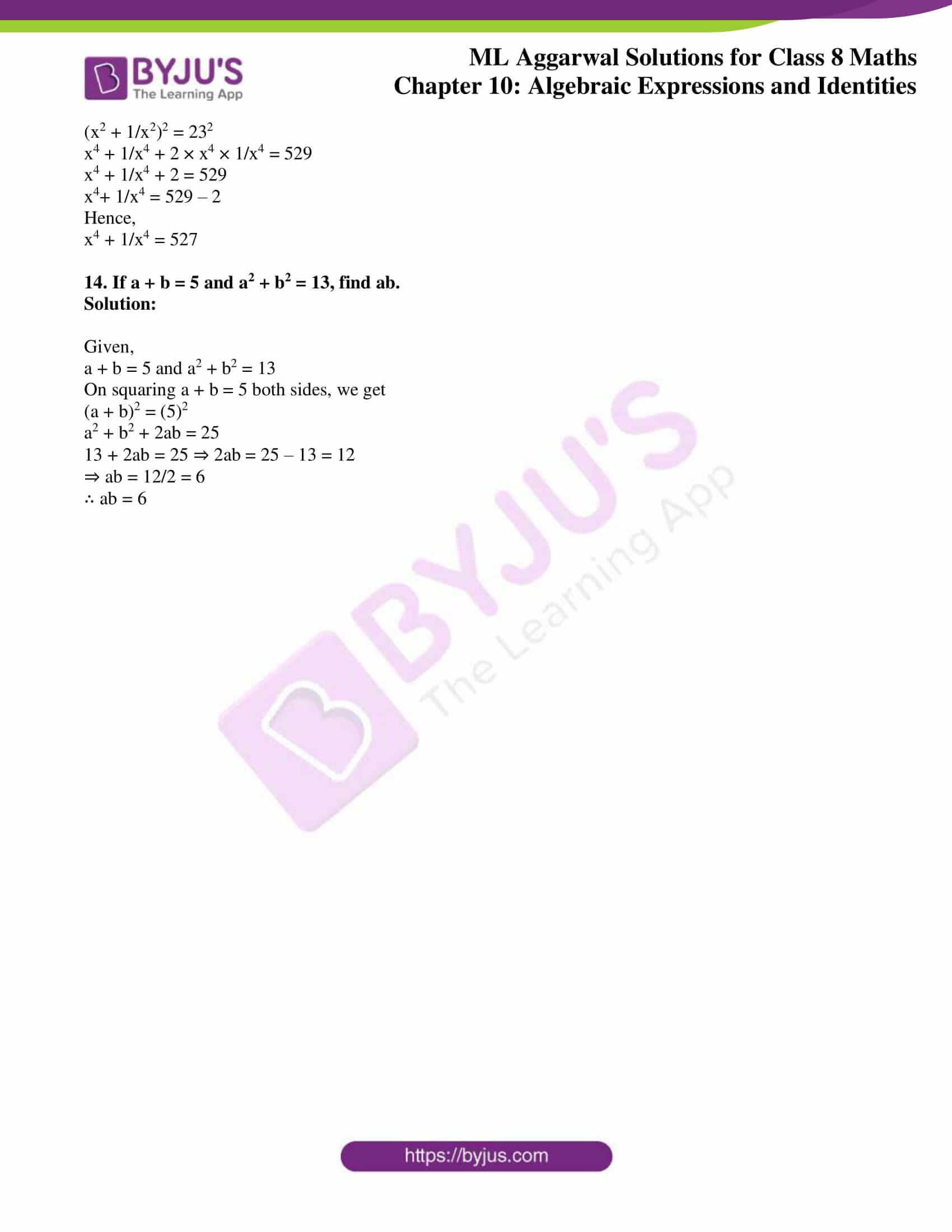### Access answers to ML Aggarwal Solutions for Class 8 Maths Chapter 10 Algebraic Expressions and Identities

Exercise 10.1

1. Identify the terms, their numerical as well as literal coefficients in each of the following expressions:
(i) 12x2yz – 4xy2
(ii) 8 + mn + nl – lm

(iii) x2/3 + y/6 – xy2

(iv) -4p + 2.3q + 1.7r
Solution: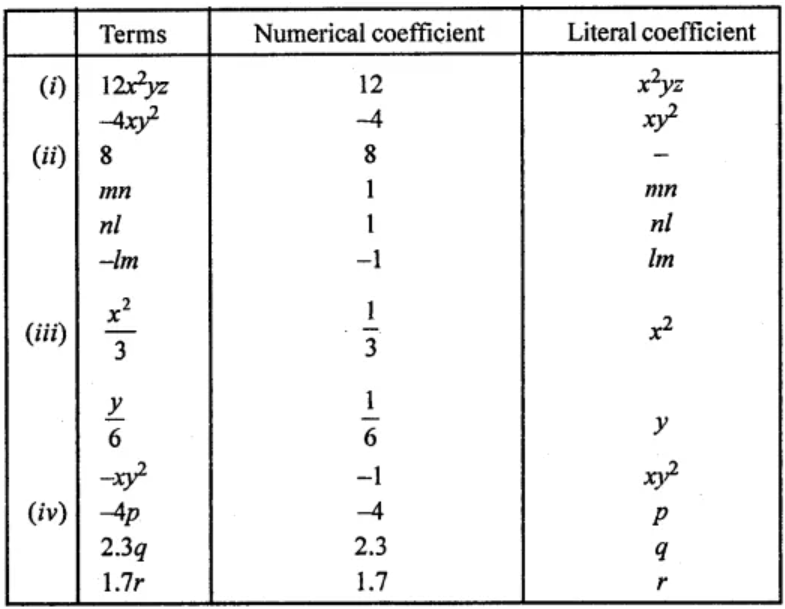2. Identify monomials, binomials, and trinomials from the following algebraic expressions :
(i) 5p × q × r2
(ii) 3x2 + y ÷ 2z
(iii) -3 + 7x2
(iv) (5a2 – 3b2 + c)/2
(v) 7x5 – 3x/y
(vi) 5p ÷ 3q – 3p2 × q2
Solution:

(i) 5p × q × r2 = 5pqr2

As this algebraic expression has only one term, its therefore a monomial.

(ii) 3x2 + y ÷ 2z = 3x2/2z + y/2z

As this algebraic expression has two terms, its therefore a binomial.

(iii) -3 + 7x2

As this algebraic expression has two terms, its therefore a binomial.

(iv)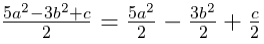As this algebraic expression has three terms, its therefore a trinomial.

(v) 7x5 – 3x/y

As this algebraic expression has two terms, its therefore a binomial.

(vi) 5p ÷ 3q – 3p2 × q2 = 5p/3q – 3p2q2

As this algebraic expression has two terms, its therefore a binomial.

3. Identify which of the following expressions are polynomials. If so, write their degrees.
(i) 2/5x4 – √3x2 + 5x – 1
(ii) 7x3 – 3/x2 + √5
(iii) 4a3b2 – 3ab4 + 5ab + 2/3
(iv) 2x2y – 3/xy + 5y3 + √3
Solution:

(i) It is a polynomial and the degree of this expression is 4.

(ii) It is not a polynomial.

(iii) It is a polynomial and the degree of this expression is 5.

(iv) It is not a polynomial.

(i) ab – bv, bv – ca, ca – ab
(ii) 5p2q2 + 4pq + 7, 3 + 9pq – 2p2q
(iii) l2 + m2 + n2, lm + mn, mn + nl, nl + lm
(iv) 4x3 – 7x2 + 9, 3x2 – 5x + 4, 7x3 – 11x + 1, 6x2 – 13x
Solution:

(i) ab – bc, bc – ca, ca – ab

On adding the expressions, we have

⇒ ab – bc + bc – ca + ca – ab = 0

(ii) 5p2q2 + 4pq + 7,3 + 9pq – 2p2q2

On adding the expressions, we have

= 5p2q2 + 4pq + 7 + 3 + 9pq – 2p2q2

= 5p2q2 – 2p2q2 + 4pq + 9pq + 7 + 3

= 3p2q2 + 13pq + 10

(iii) l2 + m2 + n2, lm + mn, mn + nl, nl + lm

On adding the expressions, we have

= l2 + m2 + n2 + lm + mn + mn + nl + nl + lm

= l2 + m2 + n2 + 2lm + 2mn + 2nl

(iv) 4x3 – 7x2 + 9, 3x2 – 5x + 4, 7x3 – 11x + 1, 6x2 – 13x

On adding the expressions, we have

= 4x3 – 7x2 + 9 + 3x2 – 5x + 4 + 7x3 – 112 + 1 + 6x2 – 13x

= 4x2 + 7x3 – 7x2 + 3x2 + 6x2 – 5x – 11x – 13x + 9 + 4 + 1

= 11x3 – 2x2 – 29x + 14

5. Subtract:
(i) 8a + 3ab – 2b + 7 from 14a – 5ab + 7b – 5
(ii) 8xy + 4yz + 5zx from 12xy – 3yz – 4zx + 5xyz
(iii) 4p2q – 3pq + 5pq2 – 8p + 7q -10 from 18 – 3p – 11q + 5pq – 2pq2 + 5p2q
Solution:

(i) Subtracting 8a + 3ab – 2b + 7 from 14a – 5ab + 7b – 5, we have

= (14a – 5ab + 7b – 5) – (8a + 3ab – 2b + 7)

= 14a – 5ab + 7b – 5 – 8a – 3ab + 2b – 7

= 6a – 8ab + 9ab – 12

(ii) Subtracting 8xy + 4yz + 5zx from 12xy – 3yz – 4zx + 5xyz, we have

= (12xy – 3yz – 4zx + 5xyz) – (8xy + 4yz + 5zx)

= 12xy – 3yz – 4zx + 5xyz – 8xy – 4yz – 5zx

= 4xy – 7yz – 9zx + 5xyz

(iii) Subtracting 4p2q – 3pq + 5pq2 – 8p + 7q – 10 from 18 – 3p – 11q + 5pq – 2pq2 + 5p2q, we have

= (18 – 3p – 11q + 5pq – 2pq2 + 5p2q) – (4p2q – 3pq + 5pq2 – 8p + 7q – 10)

= 18 – 3p – 11q + 5pq – 2pq2 + 5p2q – 7p2q + 3pq – 5pq2 + 8p – 7q + 10

= 28 + 5p – 78q + 8pq – 7pq2 + p2q

6. Subtract the sum of 3x2 + 5xy + 7y2 + 3 and 2x2 – 4xy – 3y2 + 7 from 9x2 – 8xy + 11y2
Solution:

First, adding 3x2 + 5xy + 7y2 + 3 and 2x2 – 4xy – 3y2 + 7, we have

= 3x2 + 5xy + 7y2 + 3 + 2x2 – 4xy – 3y2 + 7

= 5x2 + xy + 4y2 + 10

Now,

Subtracting 5x2 + xy + 4y2 + 10 from 9x2 – 8xy + 11y2

= (9x2 – 8xy + 11y2) – (5x2 + xy + 4y2 + 10)

= 9x2 – 8xy + 11y2 – 5x2 – xy – 4y2 – 10

= 4x2 – 9xy + 7y2 – 10

7. What must be subtracted from 3a2 – 5ab – 2b2 – 3 to get 5a2 – 7ab – 3b2 + 3a?
Solution:

From the question, its understood that we have to subtract 5a2 – 7ab – 3b2 + 3a from 3a2 – 5ab – 2b2 – 3

= 3a2 – 5ab – 2b2 – 3 – (5a2 – 7ab – 3b2 + 3a)

= 3a2 – 5ab – 2b2 – 3 – 5a2 + 7ab + 3b2 – 3a

= -2a2 + 2ab + b2 – 3a – 3

8. The perimeter of a triangle is 7p2 – 5p + 11 and two of its sides are p2 + 2p – 1 and 3p2 – 6p + 3. Find the third side of the triangle.
Solution:

Given,

Perimeter of a triangle = 7p2 – 5p + 11

And, two of its sides are p2 + 2p – 1 and 3p2 – 6p + 3

We know that,

Perimeter of a triangle = Sum of three sides of triangle

⇒ 7p2 – 5p + 11 = (p2 + 2p – 1) + (3p2 – 6p + 3) + (Third side of triangle)

7p2 – 5p + 11 = (4p2 – 4p + 2) + (Third side of triangle)

⇒ Third side of triangle = (7p2 – 5p + 11) – (4p2 – 4p + 2)

= (7p2 – 4p2) + (- 5p + 4p) + (11 – 2)

= 3p2 – p + 9

Thus, the third side of the triangle is 3p2 – p + 9.

Exercise 10.2

1. Find the product of:
(i) 4x3 and -3xy
(ii) 2xyz and 0
(iii) –(2/3)p2q, (3/4)pq2 and 5pqr
(iv) -7ab, -3a3 and –(2/7)ab2
(v) –½x2 – (3/5)xy, (2/3)yz and (5/7)xyz
Solution:

Product of:

(i) 4x3 and -3xy = 4x3 × (-3xy) = -12x3+1 y = -12x4y

(ii) 2xyz and 0 = 2xyz × 0 = 0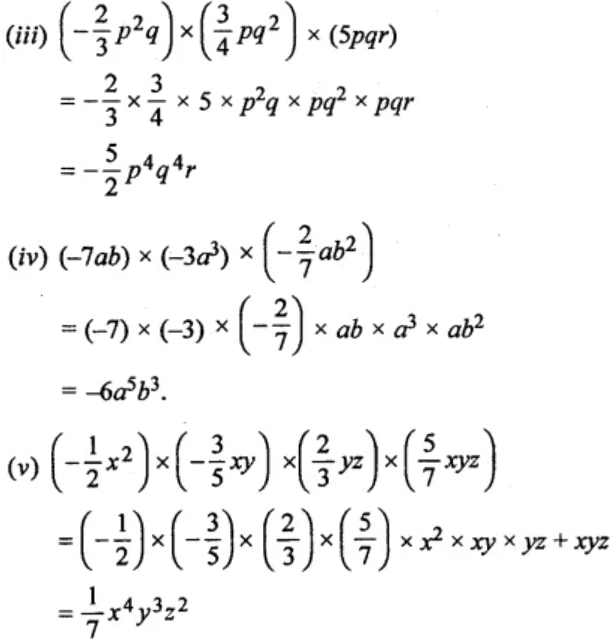2. Multiply:
(i) (3x – 5y + 7z) by – 3xyz
(ii) (2p2 – 3pq + 5q2 + 5) by – 2pq
(iii) (2/3a2b – 4/5ab2 + 2/7ab + 3) by 35ab
(iv) (4x2 – 10xy + 7y2 – 8x + 4y + 3) by 3xy
Solution:

(i) – 3xyz × (3x – 5y + 7z)

= (- 3xyz) × 3x + (- 3xyz) × (- 5y) + (- 3xyz) × (7z)

= – 9x2yz + 15xyz2 – 21xyz2

(ii) -2pq × (2p2 – 3pq + 5q2 + 5)

= (-2pq) × 2p2 + (-2pq) × (-3pq) + (- 2pq) × (5q2) + (-2pq) × 5

= -4p3q + 6p2q2 – 10pq3 – 10pq

(iii)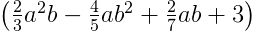by 35ab

= (2/3)a2b × 35ab – (4/5)ab2 × 35ab + (2/7)ab × 35ab + 3 × 35ab

= (70/3)a3b2 – 28a2b3 + 10a2b2 + 105ab

(iv) (4x2 – 10xy + 7y2 – 8x + 4y + 3) by 3xy

= 4x2 × 3xy – 10xy × 3xy + 7y2 × 3xy – 8x × 3xy + 4y × 3xy + 3 × 3xy

= 12x3y – 30x2y2 + 21xy3 – 24x2y + 12xy2 + 9xy

3. Find the areas of rectangles with the following pairs of monomials as their lengths and breadths respectively:
(i) (p2q, pq2)
(ii) (5xy, 7xy2)
Solution:

(i) Given, sides of a rectangle are p2q and pq2

Hence,

Area = p2q × pq2 = p2+1 × q2+1 = p3q3

(ii) Given, sides are 5xy and 7xy2

Hence,

Area = 5xy × 7xy2 = 35x1+1 × y1+2 = 35x2y3

4. Find the volume of rectangular boxes with the following length, breadth and height respectively:
(i) 5ab, 3a2b, 7a4b2
(ii) 2pq, 4q2, 8rp
Solution:

Given are the length, breadth and height of a rectangular box:

(i) 5ab, 3a2b, 7a4b2

∴ Volume = Length × breadth × height

= 5ab × 3a2b × 7a4b2

= 5 × 3 × 7 × a1+2+4 × b1+1+2

= 105a7b4

(ii) 2pq, 4q2, 8rp

∴ Volume = Length × breadth × height

= 2pq × 4q2 × 8rp

= 2 × 4 × 8 × p1+1 × q1+2 × r

= 64p2q3r

5. Simplify the following expressions and evaluate them as directed:
(i) x2(3 – 2x + x2) for x = 1; x = -1; x = 2/3 and x = –1/2
(ii) 5xy(3x + 4y – 7) – 3y(xy – x2 + 9) – 8 for x = 2, y = -1
Solution:

(i) x2(3 – 2x + x2)

For x = 1; x = -1; x = 2/3 and x = –1/2

x2(3 – 2x + x2) = 3x2 – 2x3 + x4

(a) For x = 1

3x2 – 2x3 + x4 = 3(1)2 – 2(1 )3 + (1)4

= 3 × 1 – 2 × 1 + l

= 3 – 2 + 1 = 2

(b) For x = -1

3x2 – 2x3 + x4 = 3(-1)2 – 2(-1)3 + (-1)4

= 3 × 1 – 2 × (-1) + 1

= 3 + 2 + 1 = 6

(c) For x = 2/3

3x2 – 2x3 + x4 = 3(2/3)2 – 2(2/3)3 + (2/3)4

= 3 × (4/9) – 2 × (8/27) + (16/81)

= (4/3) – (16/27) + (16/81)

= (108 – 48 + 16)/81

= (124 – 48)/81

= 76/81

(d) For x = -1/2

3x2 – 2x3 + x4 = 3(-1/2)2 – 2(-1/2)3 + (-1/2)4

= 3 × (1/4) – 2 × (-1/8) + (1/16)

= (3/4) + ¼ + (1/16)

= (12 + 4 + 1)/16

= 17/16

(ii) 5xy(3x + 4y – 7) – 3y(xy – x2 + 9) – 8

= 15x2y + 20xy2 – 35xy – 3xy2 + 3 x2y – 21y – 8

= 18x2y + 17xy2 – 35xy – 27y – 8

When x = 2, y = -1, we have

= 18(2)2 × (-1) + 17(2) (-1)2 – 35(2) (-1) – 27(-1) – 8

= 18 × 4 × (-1) + 17 × 2 × 1 – 35 × 2 × (-1) – 27 × (-1) – 8

= -74 + 34 + 70 + 27 – 8

= 131 – 80 = 51

(i) 4p(2 – p2) and 8p3 – 3p
(ii) 7xy(8x + 2y – 3) and 4xy2(3y – 7x + 8)
Solution:

(i) 4p(2 – p2) and 8p3 – 3p

= 8p – 4p3 + 8p3 – 3p

= 5p + 4p3

= 4p3 + 5p

(ii) 7xy(8x + 2y – 3) and 4xy2(3y – 7x + 8)

= 56x2y + 14xy2 – 21xy + 12xy3 – 28x2y2 + 32xy2

= 12xy3 – 28x2y2 + 56x2y +46xy2 – 21xy

7. Subtract:
(i) 6x(x – y + z)- 3y(x + y – z) from 2z(-x + y + z)
(ii) 7xy(x2 -2xy + 3y2) – 8x(x2y – 4xy + 7xy2) from 3y(4x2y – 5xy + 8xy2)
Solution:

Subtracting,

(i) 6x(x – y + z) – 3y(x + y – z) from 2z(-x + y + z)

⇒ 6x2 – 6xy + 6xz – 3xy – 3y2 + 3yz from – 2xz + 2yz + 2z2

= (-2xz + 2yz + 2z2) – (6x2 – 6xy + 6xz – 3xy – 3y2 + 3yz)

= – 2xz + 2yz + 2z2 – 6x2 + 6xy – 6xz + 3xy + 3y2 – 3yz

= 9xy – yz – 8zx – 6x2 + 3y2 + 2z2

(ii) 7xy(x2 – 2xy + 3y2) – 8x(x2y – 4xy + 7xy2) from 3y(4x2y – 5xy + 8xy2)

⇒ 7x3y – 14x2y2 + 21xy3 – 8x3y + 32x2y – 56x2y2 from 12x2y2 – 15xy2 + 24xy3

= (12x2y2 – 15xy2 + 24xy3) – (7x3y – 14x2y2 + 21xy3 – 8x3y + 32x2y – 56x2y2

= 12x2y2 – 15xy2 + 24xy3 – 7x3y + 14x2y2 – 12xy3 + 8x3y – 32x2y + 56x2y2

= 82x2y2 + 3xy3 + x3y – 15xy2 – 32x2y

Exercise 10.3

1. Multiply:
(i) (5x – 2) by (3x + 4)
(ii) (ax + b) by (cx + d)
(iii) (4p – 7) by (2 – 3p)
(iv) (2x2 + 3) by (3x – 5)
(v) (1.5a – 2.5b) by (1.5a + 2.56)
(vi)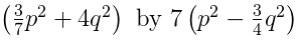Solution:

(i) (5x – 2) by (3x + 4)

= (5x – 2) × (3x + 4)

= 5x (3x + 4) – 2 (3x + 4)

= 15x2 + 20x – 6x – 8

= 15x2+ 14x – 8

(ii) (ax + b) by (cx + d)

= (ax + b) × (cx + d)

= ax (cx + d) + b (cx + d)

= acx2 + adx + bcx + bd

(iii) (4p – 7) by (2 – 3p)

= (4p – 7) × (2 – 3p)

= 4p(2 – 3p) -7(2 – 3p)

= 8p – 12p2 – 14 + 21p

= 29p – 12p2 – 14

(iv) (2x2 + 3) by (3x – 5)

= (2x2 + 3) (3x – 5)

= 2x2(3x – 5) + 3(3x – 5)

= 6x3 – 10x2 + 9x – 15

(v) (1.5a – 2.5b) by (1.5a + 2.5b)

= (1.5a – 2.5b) (1.5a + 2.5b)

= 1.5a(1.5 + 2.5b) – 2.5b(1.5a + 2.5b)

= 2.25a2 + 3.75ab – 3.75a6 – 6.25b2

= 2.25a2 – 6.25b2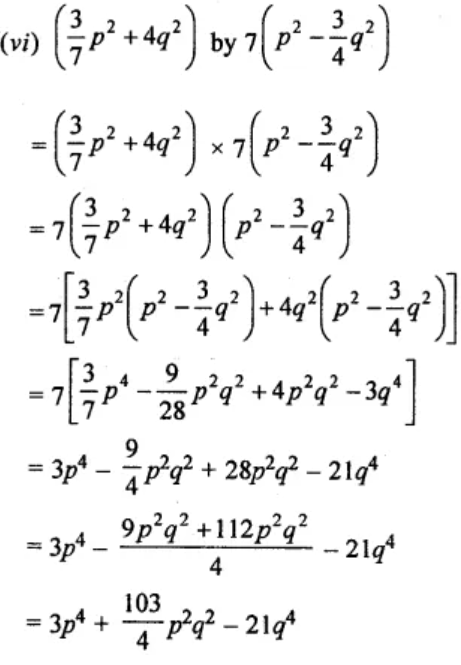2. Multiply:
(i) (x – 2y + 3) by (x + 2y)
(ii) (3 – 5x + 2x2) by (4x – 5)

Solution:

(i) (x – 2y + 3) by (x + 2y)

= (x – 2y + 3) × (x + 2y)

= x (x + 2y) – 2y(x + 2y) + 3 (x + 2y)

= x2 + 2xy – 2xy – 4y2 + 3x + 6y

= x2 – 4y2 + 3x + 6y

(ii) (3 – 5x + 2x2) by (4x – 5)

= (4x – 5) (3 – 5x + 2x2)

= 4x(3 – 5x + 2x2) – 5(3 – 5x + 2x2)

= 12x – 20x2 + 8x3 – 15 + 25x – 10x2

= 8x3 – 30x2 + 37x – 15

3. Multiply:
(i) (3x2 – 2x – 1) by (2x2 + x – 5)
(ii) (2 – 3y – 5y2) by (2y – 1 + 3y2)
Solution:

(i) (3x2 – 2x – 1) by (2x2 + x – 5)

= (3x2 – 2x – 1) (2x2 + x – 5)

= 3x2(2x2 + x – 5) – 2x(2x2 + x – 5) -1(2x2 + x – 5)

= 6x4 + 3x3 – 15x2 – 4x3 – 2x2 + 10x – 2x2 – x + 5

= 6x4 – x3 – 19x2 + 9x + 5

(ii) (2 – 3y – 5y2) by (2y- 1 + 3y2)

= (2 – 3y – 5y2) × (2y- 1 + 3y2)

= 2(2y – 1 + 3y2 ) – 3y (2y – 1 + 3y2) -5y2(2y – 1 + 3y2)

= 4y – 2 + 6y2 – 6y2 + 3y – 9y3 – 10y3 + 5y2 – 15y4

= -15y4 – 19y3 + 5y2 + 7y – 2

4. Simplify:
(i) (x2 + 3) (x – 3) + 9
(ii) (x + 3) (x – 3) (x + 4) (x – 4)
(iii) (x + 5) (x + 6) (x + 7)
(iv) (p + q – 2r) (2p – q + r) – 4qr
(v) (p + q) (r + s) + (p – q)(r – s) – 2(pr + qs)
(vi) (x + y + z) (x – y + z) + (x + y – z) (-x + y + z) – 4zx
Solution:

(i) (x2 + 3) (x – 3) + 9

= x2 (x – 3) + 3(x – 3) + 9

= x2 – 3x2 + 3x – 9 + 9

= x3 – 3x2 + 3x

(ii) (x + 3) (x – 3) (x + 4) (x – 4)

= {(x + 3) (x – 3)} × {(x + 4) (x – 4)}

= {x (x – 3) + 3 (x – 3)} {x (x – 4) + 4 (x – 4)}

= (x2 – 3x + 3x – 9) {x2 – 4x + 4x – 16}

= (x2 – 9) (x2 – 16)

= x2 (x2 – 16) – 9 (x2 – 16)

= x4 – 16x2 – 9x2 + 144

= x4 – 25x2 + 144

(iii) (x + 5) (x + 6) (x + 7)

= {(x + 5) × (x + 6)} (x + 7)

= (x2 + 6x + 5x + 30) (x + 7)

= (x2 + 11x + 30) (x + 7)

= x(x2+ 11x + 30) + 7(x2+ 11x + 30)

= x3 + 11x2 + 30x + 7x2 + 77x + 210

= x3 + 18x2 + 107x + 210

(iv) (p + q – 2r)(2p – q + r) – 4qr

= p(2p – q + r) + q(2p – q + r) – 2r(2p – q + r) – 4qr

= 2p2 – pq + pr + 2pq – q2 + qr – 4pr + 2qr – 2r2 – 4qr

= 2p2 – q2 – 2r2 + pq – 3pr – 2qr

(v) (p + q)(r + s) + (p – q) (r – s) – 2(pr + qs)

= (pr + ps + qr + qs) + (pr – ps – qr + qs) – 2pr – 2qs

= 0

(vi) (x + y + z)(x – y + z) + (x + y – z)(-x + y + z) – 4zx

= x2 – xy + xz + xy – y2 + yz + xz – yz + z2 – x2 + xy + xz

– xy + x2 + yx + xz – yz – z2 – 4zx

= 0

5. If two adjacent sides of a rectangle are 5x2 + 25xy + 4y2 and 2x2 – 2xy + 3y2, find its area.
Solution:

Given,

The adjacent sides of a rectangle are 5x2 + 25xy + 4y2 and 2x2 – 2xy + 3y2

So,

Area of rectangle = Product of two adjacent sides

= (5x2 + 25xy + 4y2) (2x2 – 2xy + 3y2)

= 10x4– 10x3y+ 15x2y2 + 50x3y – 50x2y2 + 75xy3 + 8x2y2 – 8xy3 + 12y4

= 10x4 + 40x3y – 27x2y2 + 67xy3 + 12y4

Thus,

The area of the rectangle is 10x4 + 40x3y – 27x2y2 + 67xy3 + 12y4.

Exercise 10.4

1. Divide:
(i) – 39pq2r5 by – 24p3q3r
(ii) –3/4 a2b3 by 6/7 a3b2
Solution:

(i) – 39pq2r5 (÷) – 24p3q3r

= – 39pq2r5/ – 24p3q3r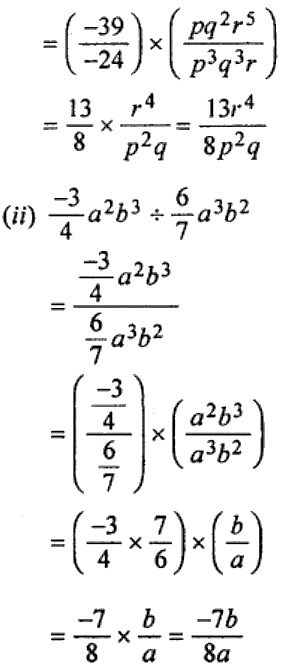2. Divide:
(i) 9x4 – 8x3 – 12x + 3 by 3x
(ii) 14p2q3 – 32p3q2 + 15pq2 – 22p + 18q by – 2p2q.
Solution: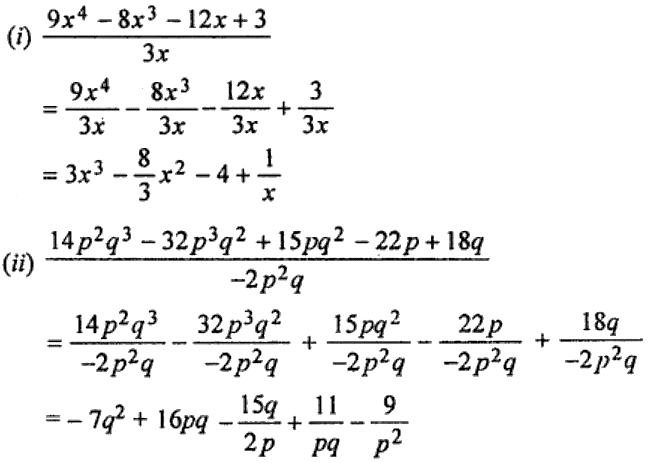3. Divide:
(i) 6x2 + 13x + 5 by 2x + 1
(ii) 1 + y3 by 1 + y
(iii) 5 + x – 2x2 by x + 1
(iv) x3 – 6x2 + 12x – 8 by x – 2
Solution:

(i) 6x2 + 13x + 5 ÷ 2x + 1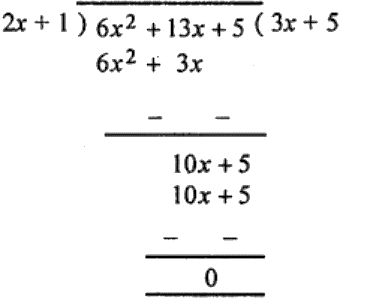∴ Quotient = 3x + 5 and remainder = 0

(ii) 1 + y3 ÷ 1 + y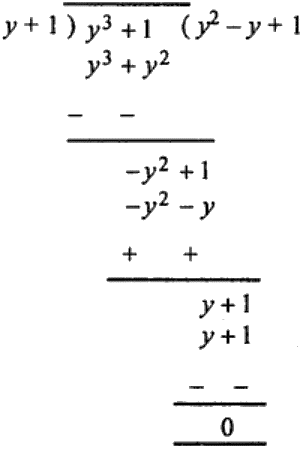∴ Quotient = y2 – y + 1 and remainder = 0

(iii) On arranging the terms of dividend in descending order of powers of x and then dividing, we get

– 2x2 + x + 5 ÷ x + 1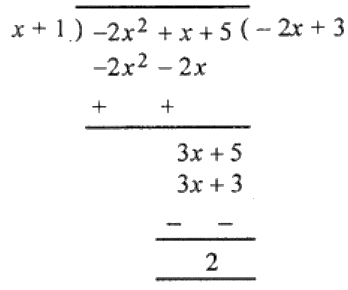∴ Quotient = – 2x + 3 and remainder = 2

(iv) x3 – 6x2 + 12x – 8 ÷ x – 2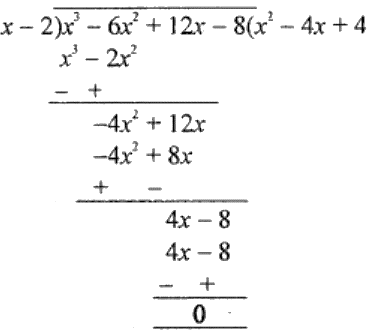∴ Quotient = x2 – 4x + 4 and remainder = 0

4. Divide:
(i) 6x3 + x2 – 26x – 25 by 3x – 7
(ii) m3 – 6m2 + 7 by m – 1
Solution:

(i) 6x3 + x2 – 26x – 25 ÷ 3x – 7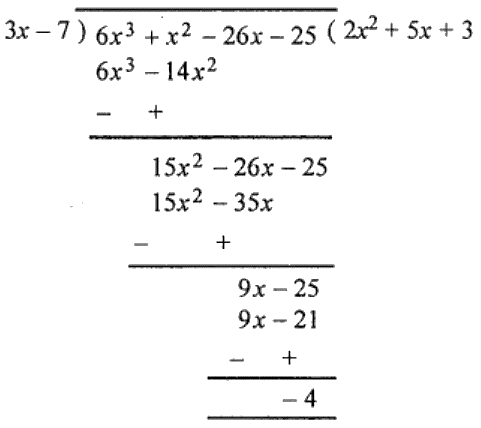∴ Quotient = 2x2 + 5x + 3 and remainder = – 4

(ii) m3 – 6m2 + 7 ÷ m – 1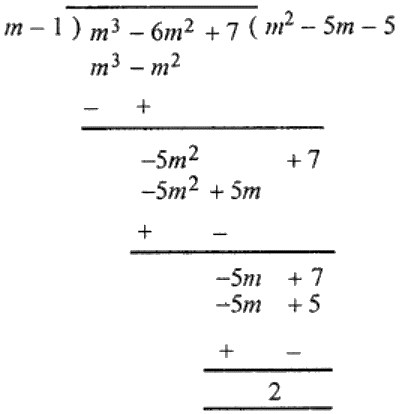∴ Quotient = m2 – 5m – 5 and remainder = 2.

5. Divide:
(i) a3 + 2a2 + 2a + 1 by a2 + a + 1
(ii) 12x3 – 17x2 + 26x – 18 by 3x2 – 2x + 5
Solution:

(i) a3 + 2a2 + 2a + 1 ÷ a2 + a + 1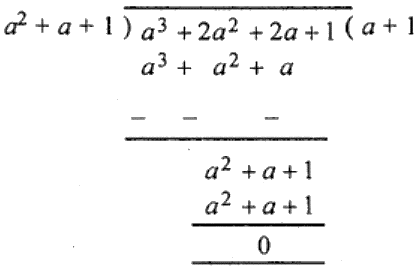∴ Quotient = a + 1 and remainder = 0.

(ii) 12x3 – 17x2 + 26x – 18 ÷ 3x2 – 2x + 5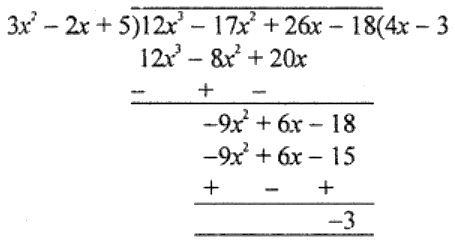∴ Quotient = 4x – 3 and remainder = -3

6. If the area of a rectangle is 8x2 – 45y2 + 18xy and one of its sides is 4x + 15y, find the length of adjacent side.
Solution:

Given,

Area of rectangle = 8x2 – 45y2 + 18xy

And, one side = 4x + 15y

∴ Second (adjacent) side = Area of rectangle/ One side

= 8x2 – 45y2 + 18xy ÷ 4x + 15y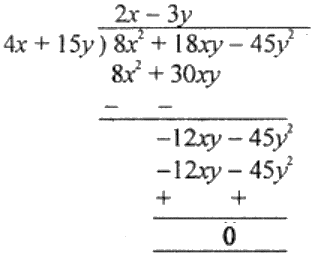Thus, length of the adjacent side is 2x – 3y.

Exercise 10.5

1. Using suitable identities, find the following products:
(i) (3x + 5) (3x + 5)
(ii) (9y – 5) (9y – 5)
(iii) (4x + 11y) (4x – 11y)
(iv) (3m/2 + 2n/3) (3m/2 – 2n/3)
(v) (2/a + 5/b) (2a + 5/b)
(vi) (p2/2 + 2/q2) (p2/2 – 2/q2)
Solution:

(i) (3x + 5) (3x + 5)

= (3x + 5)2

= (3x)2 + 2 × 3x × 5 + (5)2 [Using, (a + b)2 = a2 + 2ab + b2]

= 9x2 + 30x + 25

(ii) (9y – 5) (9y – 5)

= (9y – 5)2

= (9y)2 – 2 × 9y × 5 + (5)2 [Using, (a – b)2 = a2 – 2ab + b2]

= 81y2 – 90y + 25

(iii) (4x + 11y)(4x – 11y)

= (4x)2 – (11y)2

= 16x2 – 121y2 [Using, (a + b)(a – b) = a2 – b2]

(iv) (3m/2 + 2n/3) (3m/2 – 2n/3)

= (3m/2)2 – (2n/3)2

= 9m2/4 – 4n2/9 [Using, (a + b)(a – b) = a2 – b2]

(v) (2/a + 5/b) (2a + 5/b)

= (2/a + 5/b)2

= (2/a)2 + 2(2/a)(5/b) + (5/b)2 [Using, (a + b)2 = a2 + 2ab + b2]

= 4/a2 + 20a/b + 25/b2

(vi) (p2/2 + 2/q2) (p2/2 – 2/q2)

= (p2/2)2 – (2/q2)2 [Using, (a + b)(a – b) = a2 – b2]

= p4/4 – 4/q4

2. Using the identities, evaluate the following:
(i) 812
(ii) 972
(iii) 1052
(iv) 9972
(v) 6.12
(vi) 496 × 504
(vii) 20.5 × 19.5
(viii) 9.62
Solution:

(i) (81)2 = (80 + 1)2

= (80)2 + 2 × 80 × 1 + (1)2  [Using, (a + b)2 = a2 + 2ab + b2]

= 6400 + 160+ 1

= 6561

(ii) (97)2 = (100 – 3)2

= (100)2 – 2 × 100 × 3 + (3)2  [Using, (a – b)2 = a2 – 2ab + b2]

= 10000 – 600 + 9

= 10009 – 600

= 9409

(ii) (105)2 = (100 + 5)2

= (100)2 + 2 × 100 × 5 + (5)2  [Using, (a + b)2 = a2 + 2ab + b2]

= 10000+ 1000 + 25

= 11025

(iv) (997)2 = (1000 – 3)2

= (1000)2 – 2 × 1000 × 3 + (3)2  [Using, (a – b)2 = a2 – 2ab + b2]

= 1000000 – 6000 + 9

= 1000009 – 6000

= 994009

(v) (6.1)2 = (6 + 0.1)2

= (6)2 + 2 × 6 × 0.1 +(0.1)2  [Using, (a + b)2 = a+ 2ab + b2]

= 36 + 1.2 + 0.01

= 37.21

(vi) 496 × 504

= (500 – 4) (500 + 4) [Using, (a + b) (a – b) = a2 – b2]

= (500)2 – (4)2

= 250000 – 16

= 249984

(vii) 20.5 × 19.5

= (20 + 0.5) (20 – 0.5) [Using, (a + b) (a – b) = a2 – b2]

= (20)2 – (0.5)2

= 400 – 0.25

= 399.75

(viii) (9.6)2 = (10 – 0.4)2

= (10)2 – 2 × 10 × 0.4 + (0.4)2  [Using, (a – b)2 = a2 – 2ab + b2]

= 100 – 8.0 + 0.16

= 92.16

3. Find the following squares, using the identities:
(i) (pq + 5r)2 (ii) (5a/2 – 3b/5)2

(iii) (√2a + √3b)2 (iv) (2x/3y – 3y/2x)2
Solution:

(i) (pq + 5r)2

= (pq)2 + 2 × pq × 5r + (5r)2  [Using, (a + b)2 = a2 + 2ab + b2]

= p2q2 + 10pqr + 25r2

(ii) (5a/2 – 3b/5)2

= (5a/2)2 – 2 × (5a/2) × (-3b/5) + (3b/5)2 [Using, (a – b)2 = a2 – 2ab + b2]

= 25a2/4 – 3ab + 9b2/25

(iii) (√2a + √3b)2

= (√2a)2 + 2 × √2a × √3b + (√3b)2 [Using, (a + b)2 = a2 + 2ab + b2]

= 2a2 + 2√6ab + 3b2

(iv) (2x/3y – 3y/2x)2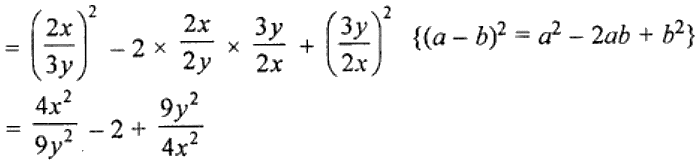4. Using the identity, (x + a) (x + b) = x2 + (a + b)x + ab, find the following products:
(i) (x + 7) (x + 3)
(ii) (3x + 4) (3x – 5)
(iii) (p2 + 2q) (p2 – 3q)
(iv) (abc + 3) (abc – 5)
Solution:

(i) (x + 7) (x + 3)

= (x)2 + (7 + 3)x + 7 × 3

= x2 + 10x + 21

(ii) (3x + 4) (3x – 5)

= (3x)2 + (4 – 5) (3x) + 4 × (-5)

= 9x2 – 3x – 20

(iii) (P2 + 2q)(p2 – 3q)

= (p2)2 + (2q – 3q)p2 + 2q × (-3q)

= p4 – p2q – 6pq

(iv) (abc + 3) (abc – 5)

= (abc)2 + (3 – 5)abc + 3 × (-5)

= a2b2c2 – 2abc – 15

5. Using the identity, (x + a) (x + b) = x2 + (a + b)x + ab, evaluate the following:
(i) 203 × 204
(ii) 8.2 × 8.7
(iii) 107 × 93
Solution:

(i) 203 × 204

= (200 + 3) (200 + 4)

= (200)2 + (3 + 4) × 200 + 3 × 4

= 40000 + 1400 + 12

= 41412

(ii) 8.2 × 8.7

= (8 + 0.2) (8 + 0.7)

= (8)2 + (0.2 + 0.7) × 8 + 0.2 × 0.7

= 64 + 8 × (0.9) + 0.14

= 64 + 7.2 + 0.14

= 71.34

(iii) 107 × 93

= (100 + 7) (100 – 7)

= (100)2 + (7 – 7) × 100 + 7 × (-7)

= 10000 + 0 – 49

= 9951

6. Using the identity a2 – b2 = (a + b) (a – b), find
(i) 532 – 472
(ii) (2.05)2 – (0.95)2
(iii) (14.3)2 – (5.7)2
Solution:

(i) 532 – 472

= (50 + 3) (50 – 3)

= (50)2 – (3)2

= 2500 – 9

= 2491

(ii) (2.05)2 – (0.95)2

= (2.05 + 0.95) (2.05 – 0.95)

= 3 × 1.10

= 3.3

(iii) (14.3)2 – (5.7)2

= (14.3 + 5.7) (14.3 – 5.7)

= 20 × 8.6

= 172

7. Simplify the following:
(i) (2x + 5y)2 + (2x – 5y)2
(ii) (7a/2 – 5b/2)2 – (5a/2 – 7b/2)2
(iii) (p2 – q2r)2 + 2p2q2r
Solution:

(i) (2x + 5y)2 + (2x – 5y)2 [Using, (a ± b)2 = a2 ± 2ab + b2]

= (2x)2 + 2 × 2x × 5y + (5y)2 + (2x)2 – 2 × 2x × 5y + (5y)2

= 4x2 + 20xy + 25y2 + 4x2 – 20xy + 25y2

= 8x2 + 50y2

(ii) (7a/2 – 5b/2)2 – (5a/2 – 7b/2)2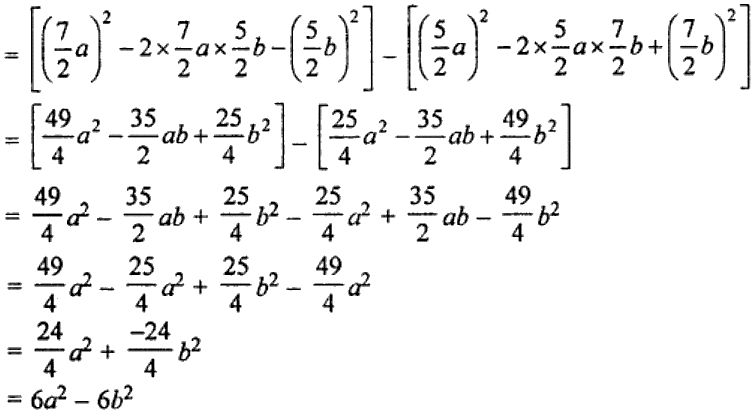(iii) (p2 – q2r)2 + 2p2q2r [Using, (a – b)2 = a2 – 2ab + b2]

= (p2)2 – 2 × p2 × q2r + (q2r)2 + 2p2q2r

= p4 – 2p2q + q4r2 + 2p2q2r

= p4 + q4r2

8. Show that:
(i) (4x + 7y)2 – (4x – 7y)2 = 112xy
(ii) (3p/7 – 7q/6)2 + pq = 9p2/49 + 49q2/36
(iii) (p – q)(p + q) + (q – r)(q + r) + (r – p) (r + p) = 0
Solution:

(i) Taking LHS, we have

LHS = (4x + 7y)2 – (4x – 7y)2 [Using, (a ± b)2 = a2 ± 2ab + b2]

= [(4x)2 + 2 × 4x × 7y + (7y)2] – [(4x)2 – 2 × 4x + 7y + (7y)2]

= (16x2 + 56xy + 49y2) – (16x2 – 56xy + 49y2)

= l6x2 + 56xy + 49y2 – 16x2 + 56xy – 49y2

= 112xy = RHS

(ii) Taking LHS, we have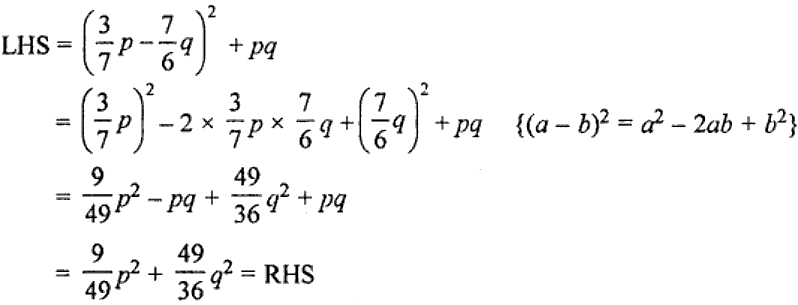(iii) Taking LHS, we have

LHS = (p – q) (p + q) + (q – r) (q + r) + (r – p)(r + p)

= p2 – q2 + q2 – r2 + r2 – p2 [Using, (a + b) (a – b) = a2 – b2]

= 0 = RHS

9. If x + 1/x = 2, evaluate:
(i) x2 + 1/x2 (ii) x4 + 1/x4
Solution:

(i) We have, x + 1/x = 2

On squaring on both sides, we get

(x + 1/x)2 = 22

x2 + 2 × x × 1/x + 1/x2 = 4

x2 + 2 + 1/x2 = 4

x2 + 1/x2 = 4 – 2

Thus,

x2 + 1/x2 = 2

(ii) Again squaring, we get

(x2 + 1/x2)2 = 22

x4 + 2 × x2 × 1/x2 + 1/x4 = 4

x4 + 2 + 1/x4 = 4

x4 + 1/x4 = 4 – 2

Thus,

x4 + 1/x4 = 2

10. If x – 1/x = 7, evaluate:
(i) x2 + 1/x2 (ii) x4 + 1/x4
Solution:

We have, x – 1/x = 7

On squaring on both sides, we get

(x – 1/x)2 = 72

x2 – 2 × x2 × 1/x2 + 1/x2 = 49

x2 – 2 + 1/x2 = 49

x2 + 1/x2 = 49 + 2

Thus,

x2 + 1/x2 = 51

(ii) Again squaring, we get

(x2 + 1/x2)2 = 512

x4 + 1/x4 + 2 × x2 × 1/x2 = 2601

x4 + 1/x4 + 2 = 2601

x4 + 1/x4 = 2601 – 2

Thus,

x4 + 1/x4 = 2599

11. If x2 + 1/x2 = 23, evaluate:
(i) x + 1/x (ii) x – 1/x
Solution:

We have, x2 + 1/x2 = 23

(i) (x + 1/x)2 = x2 + 1/x2 + 2

= 23 + 2

= 25

Taking square root on both sides, we get

(x + 1/x) = ±5

Thus, x + 1/x = 5 or -5

(ii) (x – 1/x)2 = x2 + 1/x2 – 2

= 23 – 2

= 21

Taking square root on both sides, we get

(x + 1/x) = ±√21

Thus, x + 1/x = √21 or -√21

12. If a + b = 9 and ab = 10, find the value of a2 + b2.
Solution:

Given,

a + b = 9 and ab = 10

Now, squaring a + b = 9 on both sides, we have

(a + b)2 = (9)

a2 + b2 + 2ab = 81

a2 + b2 + 2 × 10 = 81

a2 + b2 + 20 = 81

a2 + b2 = 81 – 20 = 61

∴ a2 + b2 = 61

13. If a – b = 6 and a2 + b2 = 42, find the value of
Solution:

Given

a – b = 6 and a2 + b2 = 42

a – b = 6

Now, squaring a – b = 6 on both sides, we have

(a – b)2 = (6)2

a2 + b2 – 2ab = 36

42 – 2ab = 36

2ab = 42 – 36 = 6

ab = 6/2 = 3

∴ ab = 3

14. If a2 + b2 = 41 and ab = 4, find the values of
(i) a + b
(ii) a – b
Solution:

Given, a2 + b2 = 41 and ab = 4

(i) (a + b)2 = a2 + b2 + 2ab

= 41 + 2 × 4

= 41 + 8

= 49

∴ a + b = ±7

(ii) (a – b)2 = a2 + b2 – 2ab

= 41 – 2 × 4

= 41 – 8

= 33

∴ a – b = ±√33

(i) -5x2y + 3xy2 – 7xy + 8, 12x2y – 5xy2 + 3xy – 2
(ii) 9xy + 3yz – 5zx, 4yz + 9zx – 5y, -5xz + 2x – 5xy
Solution:

(i) (-5x2y + 3xy2 – 7xy + 8) + (12x2y – 5xy2 + 3xy – 2)

= 7x2y – 2xy2 – 4xy + 6

(ii) (9xy + 3yz – 5zx) + (4yz + 9zx – 5y, -5xz + 2x – 5xy)

= 4xy + 7yz – zx + 2x – 5y

2. Subtract:
(i) 5a + 3b + 11c – 2 from 3a + 5b – 9c + 3
(ii) 10x2 – 8y2 + 5y – 3 from 8x2 – 5xy + 2y+ 5x – 3y
Solution:

(i) 5a – 3b + 11c – 2 from 3a + 5b – 9c + 3

= (3a + 5b – 9c + 3) – (5a – 3b + 11c – 2)

= 3a + 5b – 9c + 3 – 5a + 3b – 11c + 2

= -2a + 8b – 20c + 5

(ii) 10x2 – 8y2 + 5y – 3 from 8x2 – 5xy + 2y2 + 5x – 3y

= (8x2 – 5xy + 2y2 + 5x – 3y) – (10x2 – 8y2 + 5y – 3)

= 8x2 – 5xy + 2y2 + 5x – 3y – 10x2 + 8y2 – 5y + 3

= – 2x2 – 5xy + 10y2 + 5x – 8y – 3

3. What must be added to 5x2 – 3x + 1 to get 3x3 – 7x2 + 8?
Solution:

From the question, the required expression is

= (3x3 – 7x2 + 8) – (5x2 – 3x + 1)

= 3x3 – 7x2 + 8 – 5x2 + 3x – 1

= 3x3 – 12x2 + 3x + 7

4. Find the product of
(i) 3x2y and -4xy2
(ii) –(4/5)xy, (5/7)yz and –(14/9)zx
Solution:

Product of:

(i) 3x2y and -4xy2

= 3x2 × (-4xy2)

= -12x2+1 y1+2

= 12x3y3

(ii) –(4/5)xy, (5/7)yz and –(14/9)zx

= –(4/5)xy × (5/7)yz × –(14/9)zx

= –(4/5) × (5/7) × –(14/9) x2y2z2

= (8/9)x2y2z2

5. Multiply:
(i) (3pq – 4p2 + 5q2 + 7) by -7pq
(ii) (3/4x2y – 4/5xy + 5/6xy2) by – 15xyz
Solution:

(i) (3pq – 4p2 + 5q2 + 7) × (-7pq)

= -7pq × 3pq – 7pq × (-4p2) + (-7pq) (5q2) – 7pq × 7

= -21p2q2 + 28p3q – 35pq3 – 49pq

(ii) (3/4x2y – 4/5xy + 5/6xy2) × (– 15xyz)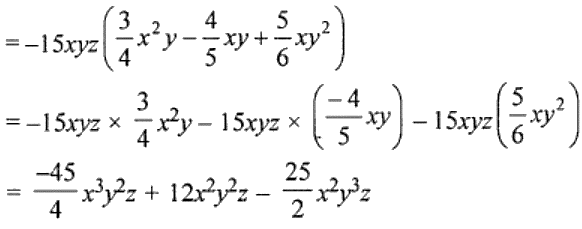6. Multiply:
(i) (5x2 + 4x – 2) by (3 – x – 4x2)
(ii) (7x2 + 12xy – 9y2) by (3x2 – 5xy + 3y2)
Solution:

(i) (5x2 + 4x – 2) × (3 – x – 4x2)

= 5x2(3 – x – 4x2) + 4x(3 – x – 4x2) – 2(3x – x – 4x2)

= 15x2 – 5x3 – 20x4 + 12x – 4x2 – 16x3 – 6x + 2x + 8x2

= -20x4 – 21x3 + 19x2 + 14x – 6

(ii) (7x2 + 12xy – 9y2) x (3x2 – 5xy + 3y2)

= 7x2(3x2 – 5xy + 3y2) + 12xy(3x2 – 5xy + 3y2) – 9y2(3x2 – 5xy + 3y2)

= 21x4 – 35x3y + 21x2y2 + 36x3y – 60x2y2 + 36xy3 – 27x2y2 + 45xy3 – 27y4

= 21x4 + x3y + 81xy3 – 66x2y2 – 27y4

7. Simplify the following expressions and evaluate them as directed:
(i) (3ab – 2a2 + 5b2) x (2b2 – 5ab + 3a2) + 8a3b – 7b4 for a = 1, b = -1
(ii) (1.7x – 2.5y) (2y + 3x + 4) – 7.8x2 – 10y for x = 0, y = 1.
Solution:

(i) (3ab – 2a2 + 5b2) × (2b2 – 5ab + 3a2) + 8a3b – 7b4

= 3ab(2b2 – 5ab + 3a2) – 2a2(2b2 – 5ab + 3a2) + 5b2(2b2 – 5ab + 3a2) + 8a3b – 7b4

= 6ab32 – 15a2b2 + 9a3b – 4a2b2 + 10a3b – 6a4 + 10b4 – 25ab3 + 15a2b2 + 8a3b – 7b4

= 27a3b – 4a2b2 – 19ab3 – 6a4 + 3b4

Putting, a = 1 and b = (-1)

= 27(1 )3 (-1) – 4(1)2 (-1)2 – 19 (1) (-1)3 – 6(1)4 + 3(-1)4

= -27 – 4 + 19 – 6 + 3

= -37 + 22

= -15

(ii) (1.7x – 2.5y) (2y + 3x + 4) – 7.8x2 – 10y

1.7x(2y + 3x + 4) – 2.5y(2y + 3x + 4) – 7.8x2 – 10y

= 3.4xy + 5.1x2 + 6.8x – 5y2 – 7.5xy – 10y – 7.8x2 – 10y

= -2.7x2 – 4.1xy – 5y2 + 6.8x – 20y

Putting, x = 0 and y = 1

= -2.7 × 0 – 4.1 × 0 × 1 – 5(1)2 + 6.8 × 0 – 20 × 1

= 0 + 0 – 5 + 0 – 20

= -25

8. Carry out the following divisions:
(i) 66pq2r3 ÷ 11qr2
(ii) (x3 + 2x2 + 3x) ÷ 2x
Solution:

(i) 66pq2r3/ 11qr2

= 6pq2-1r3-2

= 6pqr

(ii) (x3 + 2x2 + 3x)/ 2x

= x3/2x + 2x2/2x + 3x/2x

= ½ x2 + x + 3/2

9. Divide 10x4 – 19x3 + 17x2 + 15x – 42 by 2x2 – 3x + 5.
Solution:

(10x4 – 19x3 + 17x2 + 15x – 42) ÷ (2x2 – 3x + 5)

Performing long division, we have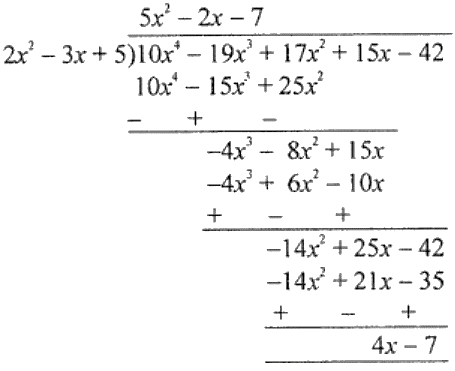Thus, Quotient = 5x2 – 2x – 7 and Remainder = 4x – 7

10. Using identities, find the following products:
(i) (3x + 4y) (3x + 4y)
(ii) (5a/2 – b) (5a/2 – b)
(iii) (3.5m – 1.5n) (3.5m + 1.5n)
(iv) (7xy – 2) (7xy + 7)
Solution:

(i) (3x + 4y) (3x + 4y)

= (3x + 4y)2

= (3x)2 + 2 × 3x × 4y + (4y)2  [Using, (a + b)2 = a2 + 2ab + b2]

= 9x2 + 24xy + 16y2

(ii) (5a/2 – b) (5a/2 – b)

= (5a/2 – b)2

= (5a/2)2 + 2 × 5a/2 × (-b) + (b)2  [Using, (a – b)2 = a2 – 2ab + b2]

= 25a2/4 – 5ab + b2

(iii) (3.5m – 1.5n) (3.5m + 1.5n)

= (3.5m)2 – (1.5n)2  [Using, (a – b)(a + b) = a2 – b2]

= 12.25m2 – 2.25n2

(iv) (7xy – 2)(7xy + 7)

= (7xy)+ (-2 + 7) × (7xy) + (-2) × 7 [Using, (x + a)(x + b) = x2 + (a + b)x + ab]

= 49x2y2 + 35xy – 14

11. Using suitable identities, evaluate the following:
(i) 1052
(ii) 972
(iii) 201 × 199
(iv) 872 – 132
(v) 105 × 107
Solution:

(i) (105)2 = (100 + 5)2

= (100)2 + 2 × 100 × 5 + (5)2 [Using, (a + b)2 = a2 + 2ab + b2]

= 10000 + 1000 + 25

= 11025

(ii) (97)2 = (100 – 3)2

= (100)2 – 2 × 100 × 3 + (3)2 [Using, (a – b)2 = a2 – 2ab + b2]

= 10000 – 600 + 9

= 10009 – 600

= 9409

(iii) 201 × 199 = (200 + 1) (200 – 1)

= (200)2 – (1)2 [Using, (a + b) (a – b) = a2 – b2]

= 40000 – 1

= 39999

(iv) 872 – 132

= (87 + 13) (87- 13) [Using, a2 – b2 = (a + b)(a – b)]

= 100 × 74

= 7400

(v) 105 × 107

= (100 + 5) (100 + 7)

= (100)2 + (5 + 7) × 100 + 5 × 7 [Using, (x + a)(x – b) = x2 + (a + b)x + ab]

= 10000 + 1200 + 35

= 11235

12. Prove that following:
(i) (a + b)2 – (a – b)2 + 4ab
(ii) (2a + 3b)2 + (2a – 3b)2 = 8a2 + 18b2
Solution:

(i) Taking the RHS, we have

RHS = (a – b)2 + 4ab

= a2– 2ab + b2 + 4ab

= a2 + 2ab + b2

= (a + b)2 = L.H.S.

(ii) Taking the LHS, we have

LHS = (2a + 3b)2 + (1a – 3b)2

= (2a)2 + 2 × 2a × 3b + (3b)2 + (2a)2 – 2 × 2a × 3b + (3b)2

= 4a2 + 12ab + 9b2 + 4a2 – 12ab + 9b2

= 8a2 + 18b2 = RHS

13. If x + 1/x = 5, evaluate
(i) x2 + 1/x2 (ii) x4 + 1/x4
Solution:

(i) We have, x + 1/x = 5

On squaring on both sides, we get

(x + 1/x)2 = 52

x2 + 1/x2 + 2 × x × 1/x = 25

x2 + 2 + 1/x2 = 25

x2 + 1/x2 = 25 – 2

Hence, x2 + 1/x2 = 23

(ii) Again, squaring x2 + 1/x2 = 23 on both sides, we get

(x2 + 1/x2)2 = 232

x4 + 1/x4 + 2 × x4 × 1/x4 = 529

x4 + 1/x4 + 2 = 529

x4+ 1/x4 = 529 – 2

Hence,

x4 + 1/x4 = 527

14. If a + b = 5 and a2 + b2 = 13, find ab.
Solution:

Given,

a + b = 5 and a2 + b2 = 13

On squaring a + b = 5 both sides, we get

(a + b)2 = (5)2

a2 + b2 + 2ab = 25

13 + 2ab = 25 ⇒ 2ab = 25 – 13 = 12

⇒ ab = 12/2 = 6

∴ ab = 6